# RD Sharma Solutions for Class 9 Maths Chapter 8 Lines and Angles

## RD Sharma Solutions Class 9 Maths Chapter 8 – Free PDF Download

RD Sharma Solutions for Class 9 Maths Chapter 8 Lines and Angles are provided here to help students in grasping the concepts within a short duration. Students can freely access RD Sharma Solutions prepared by highly experienced teachers to enhance their revision. The Chapter deals with a study of lines and angles, their different types and formulas, etc. Understanding the concepts discussed here is quite crucial and students will have to put in some amount of time to practice all questions to understand the concept in detail.

Students can download the solutions for more conceptual knowledge of the concepts. RD Sharma Solutions are so apt that students can solve any problem accurately in a short duration. Following  RD Sharma Solutions Class 9 is the main key in obtaining an idea of various methods of solving complex problems with ease. The solutions to all the textbook problems are designed in accordance with the current CBSE syllabus.

## Download PDF of RD Sharma Solutions for Class 9 Maths Chapter 8 Lines and Angles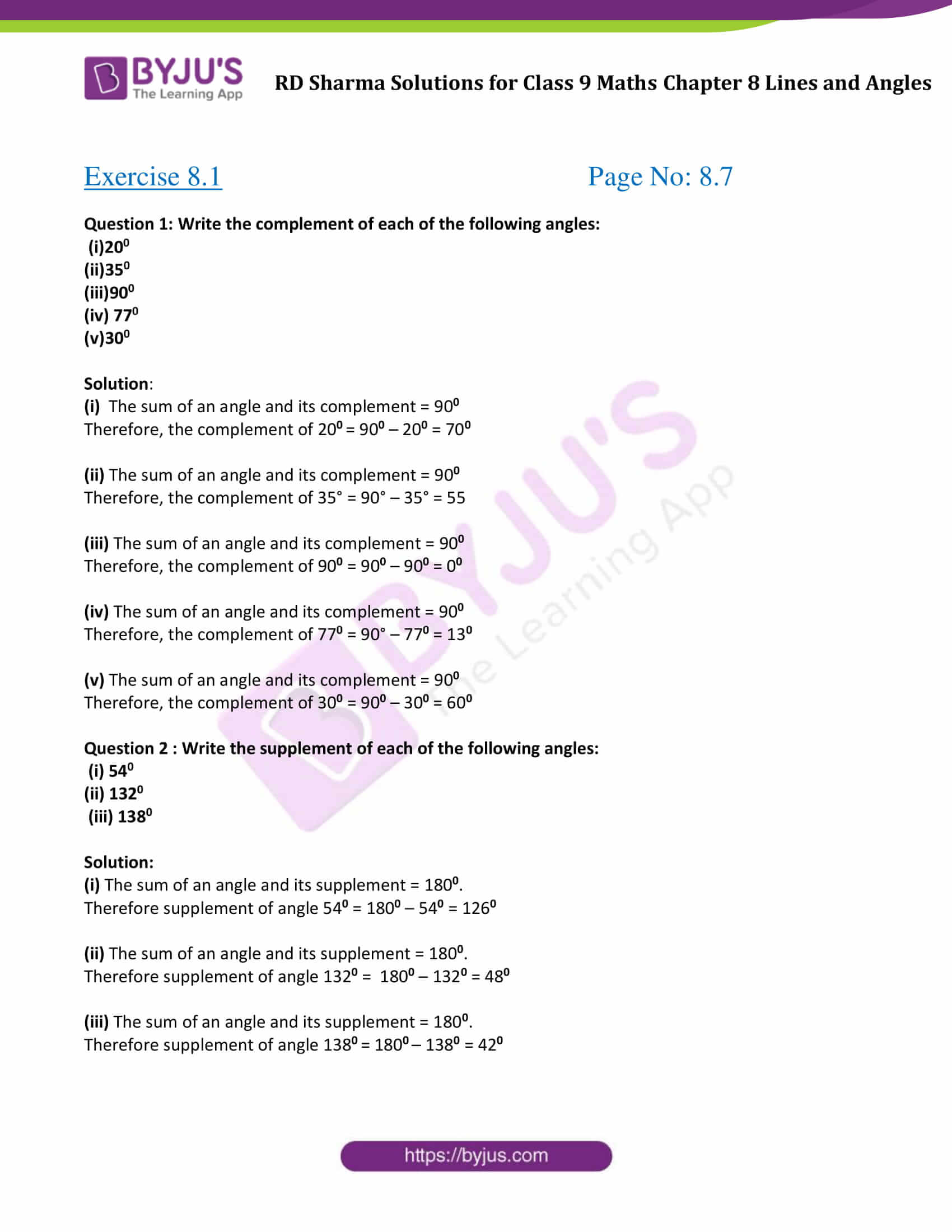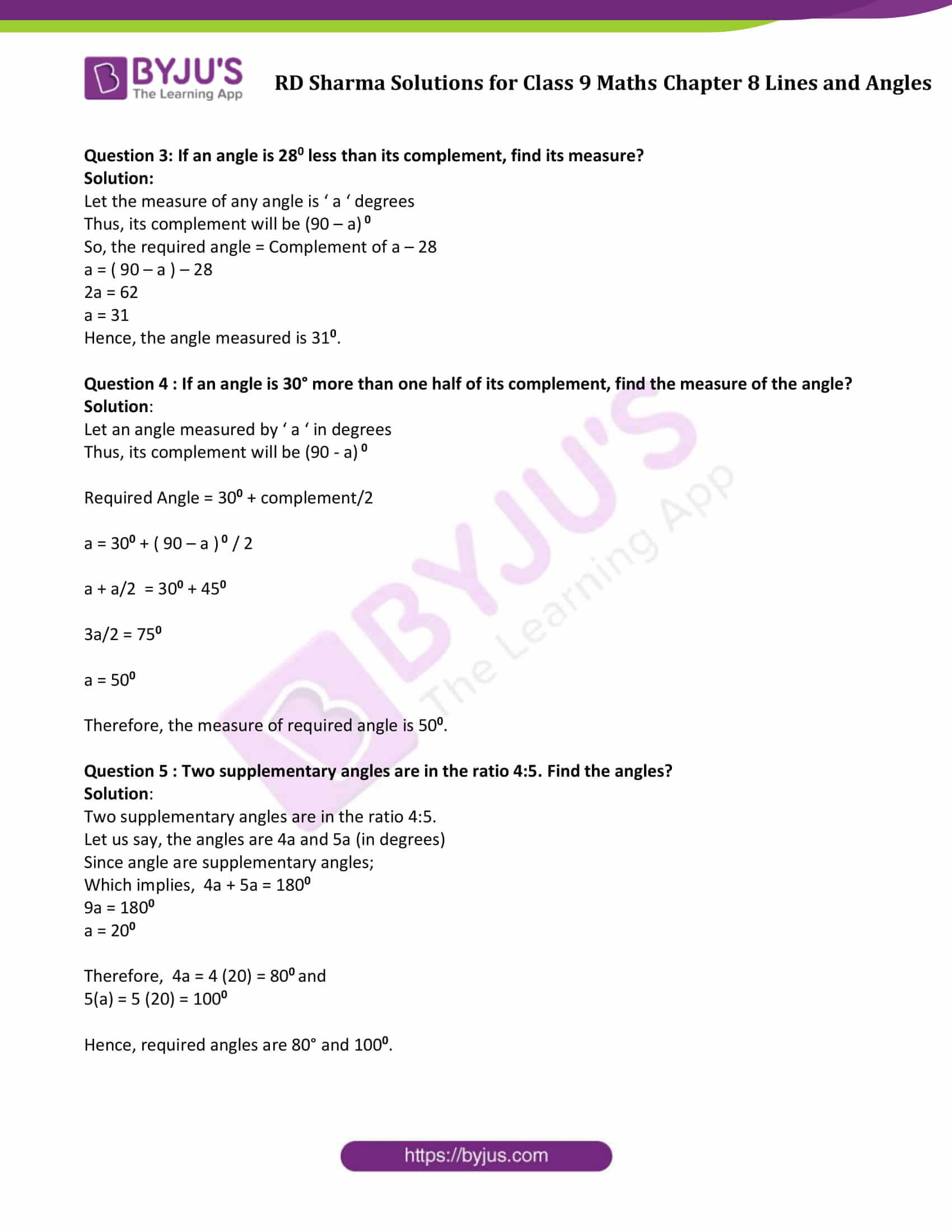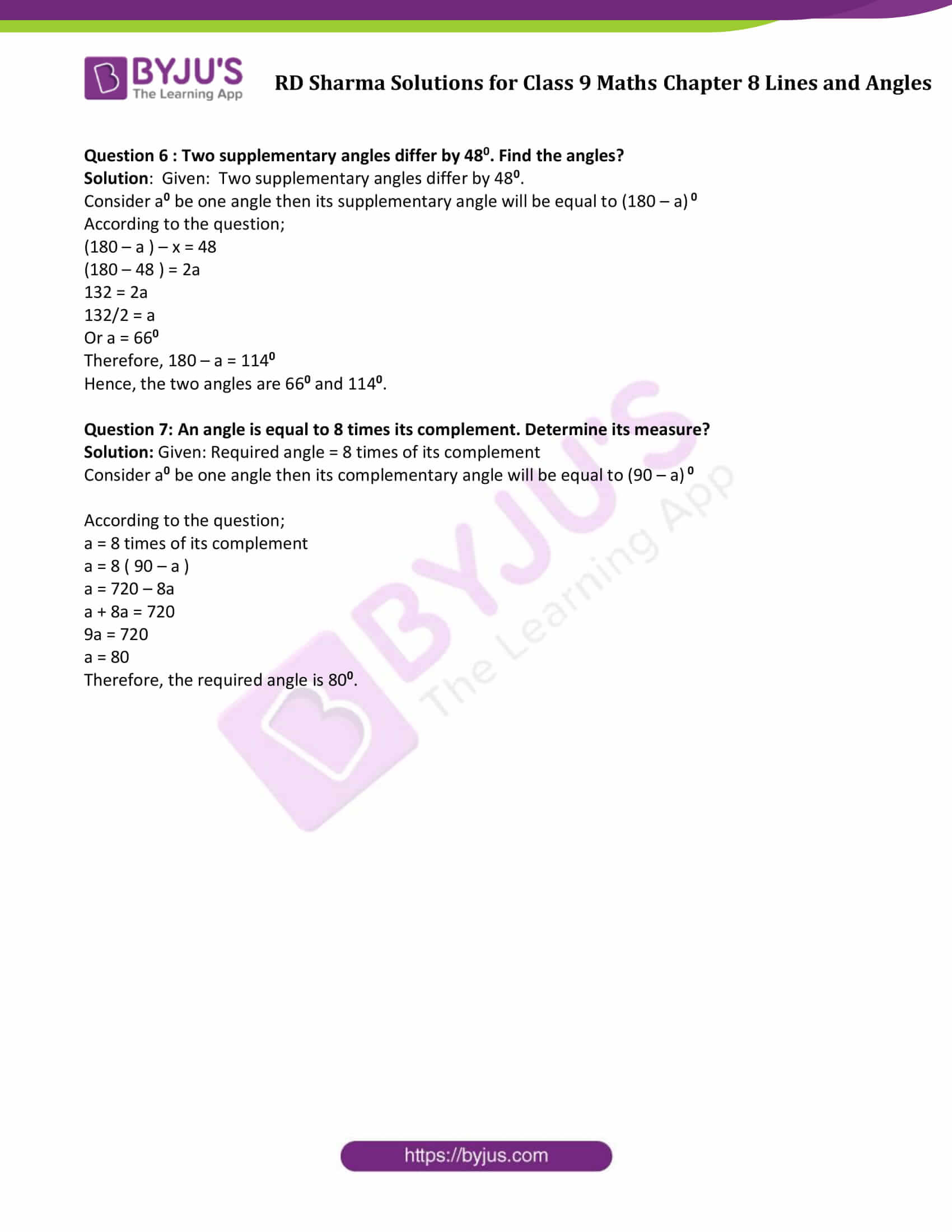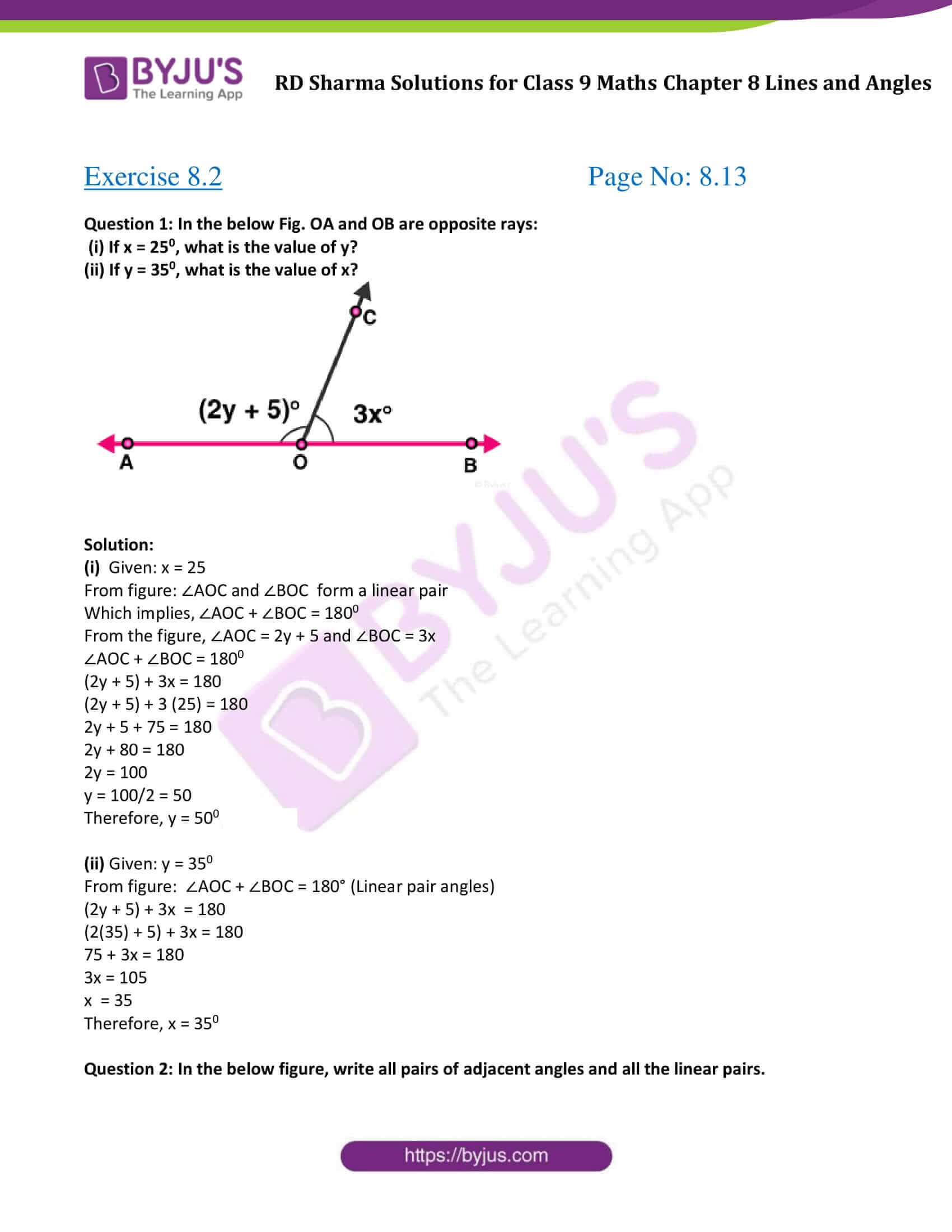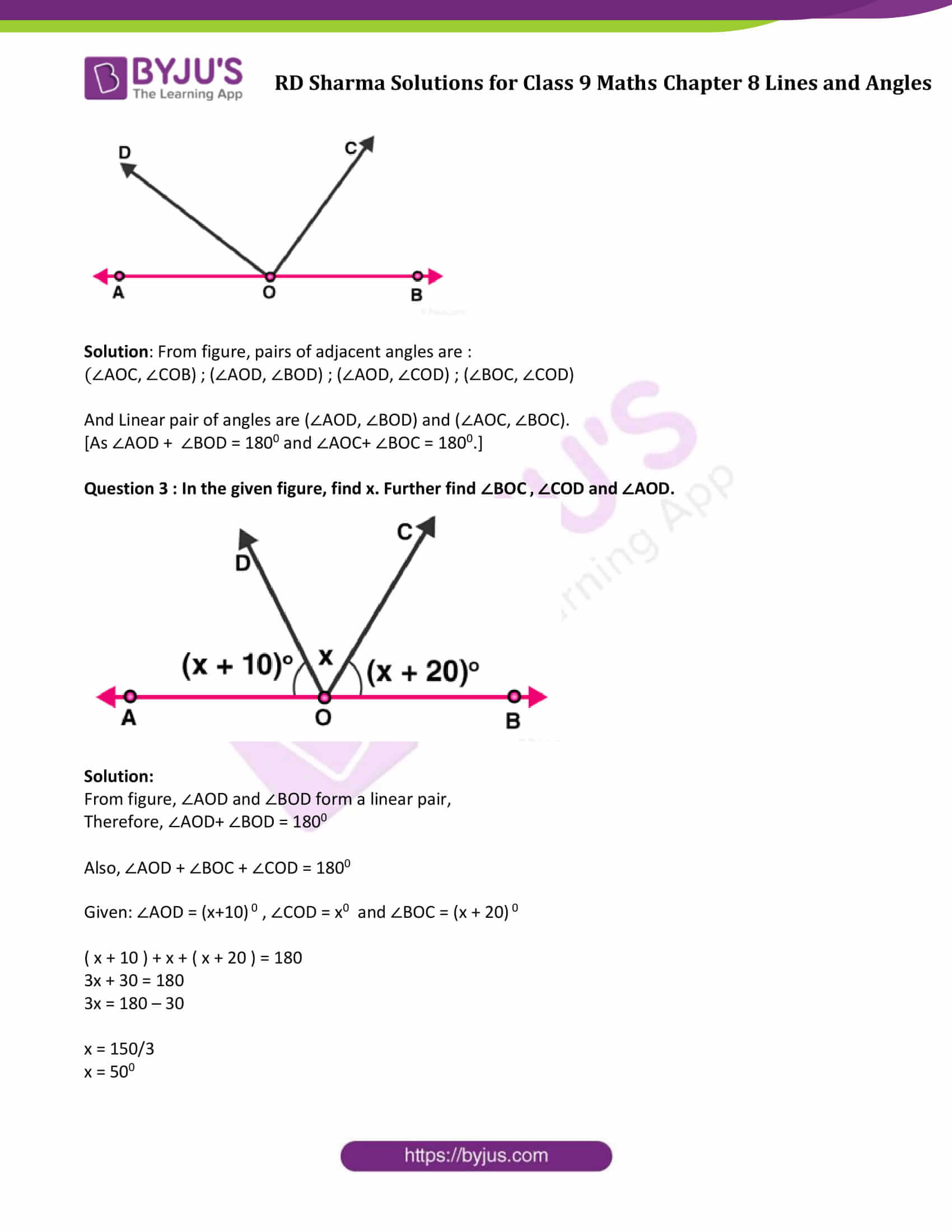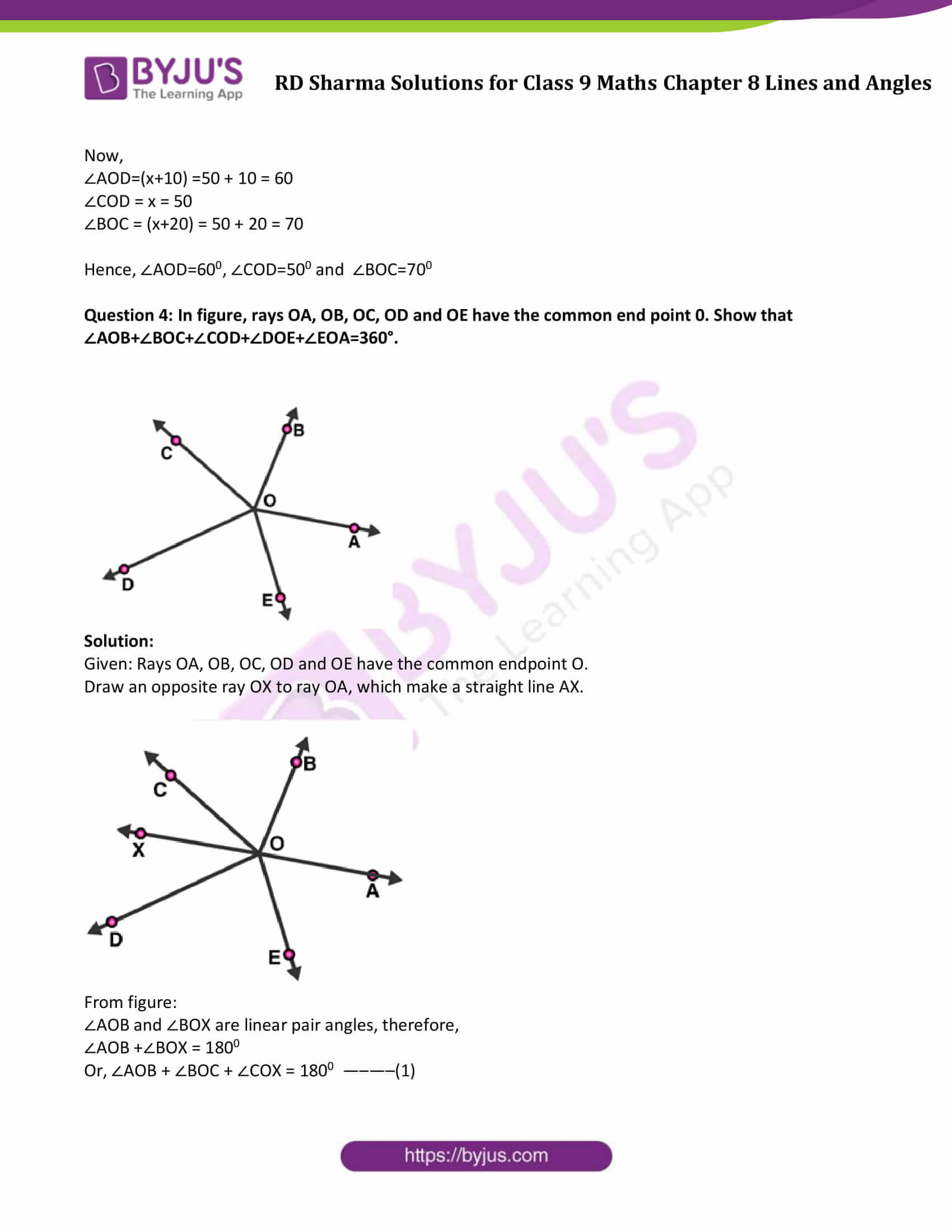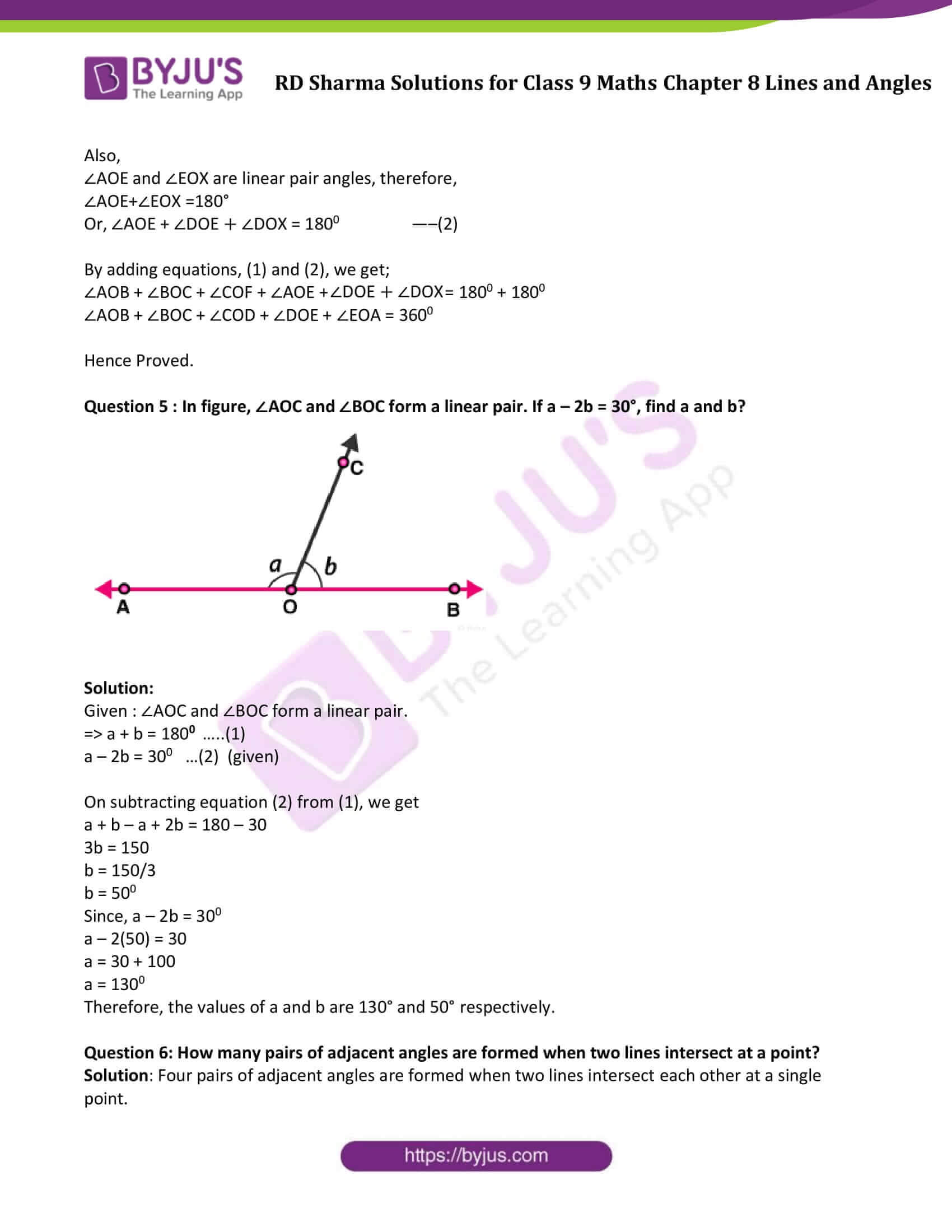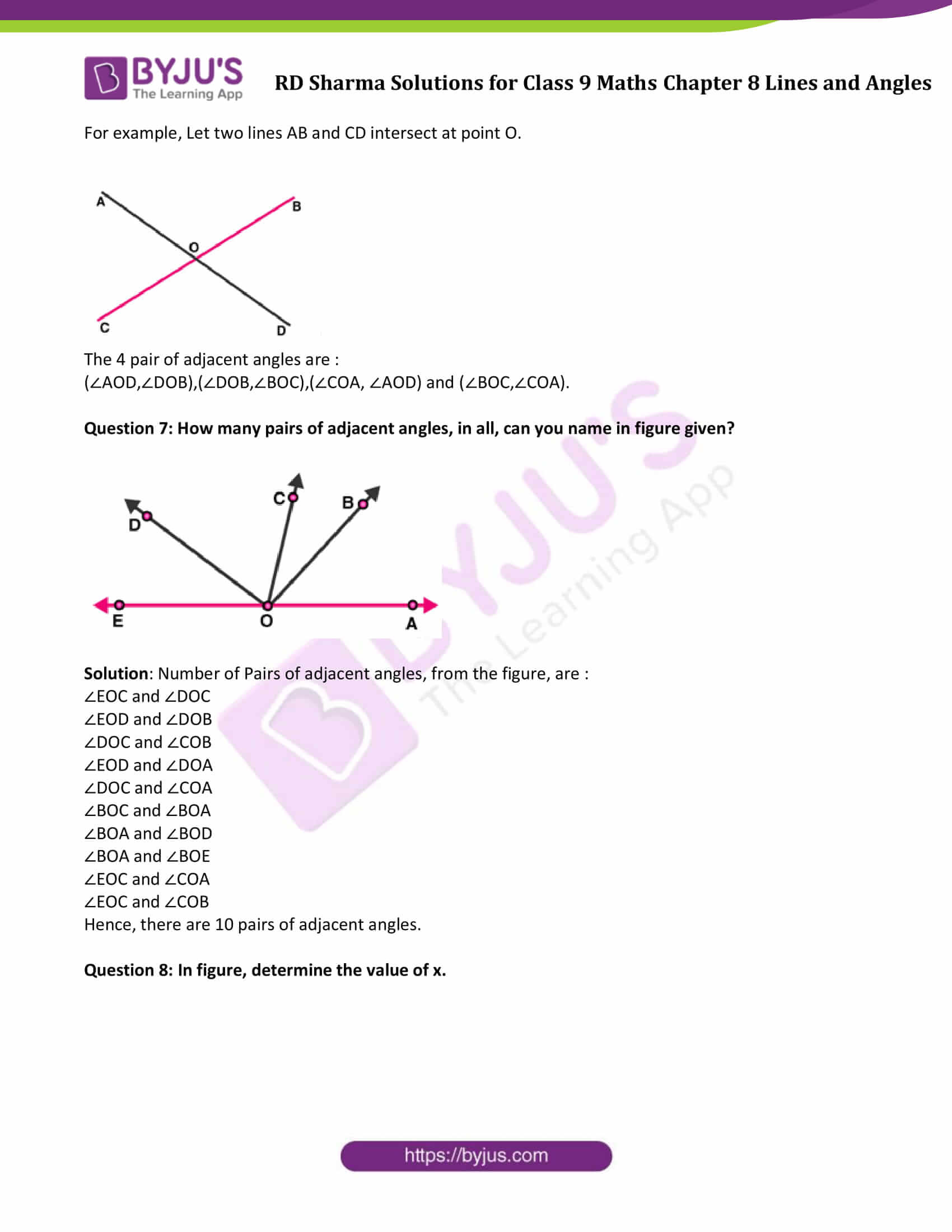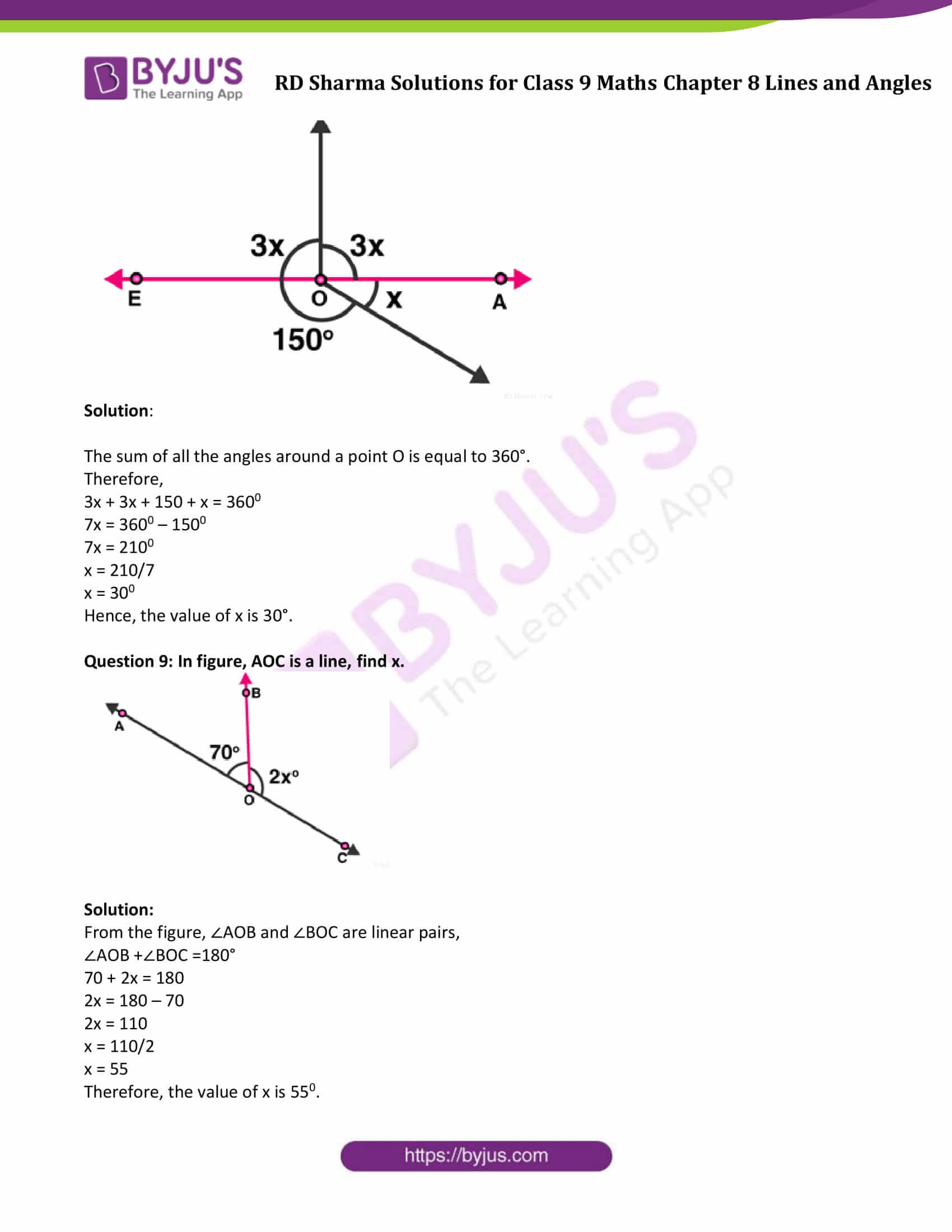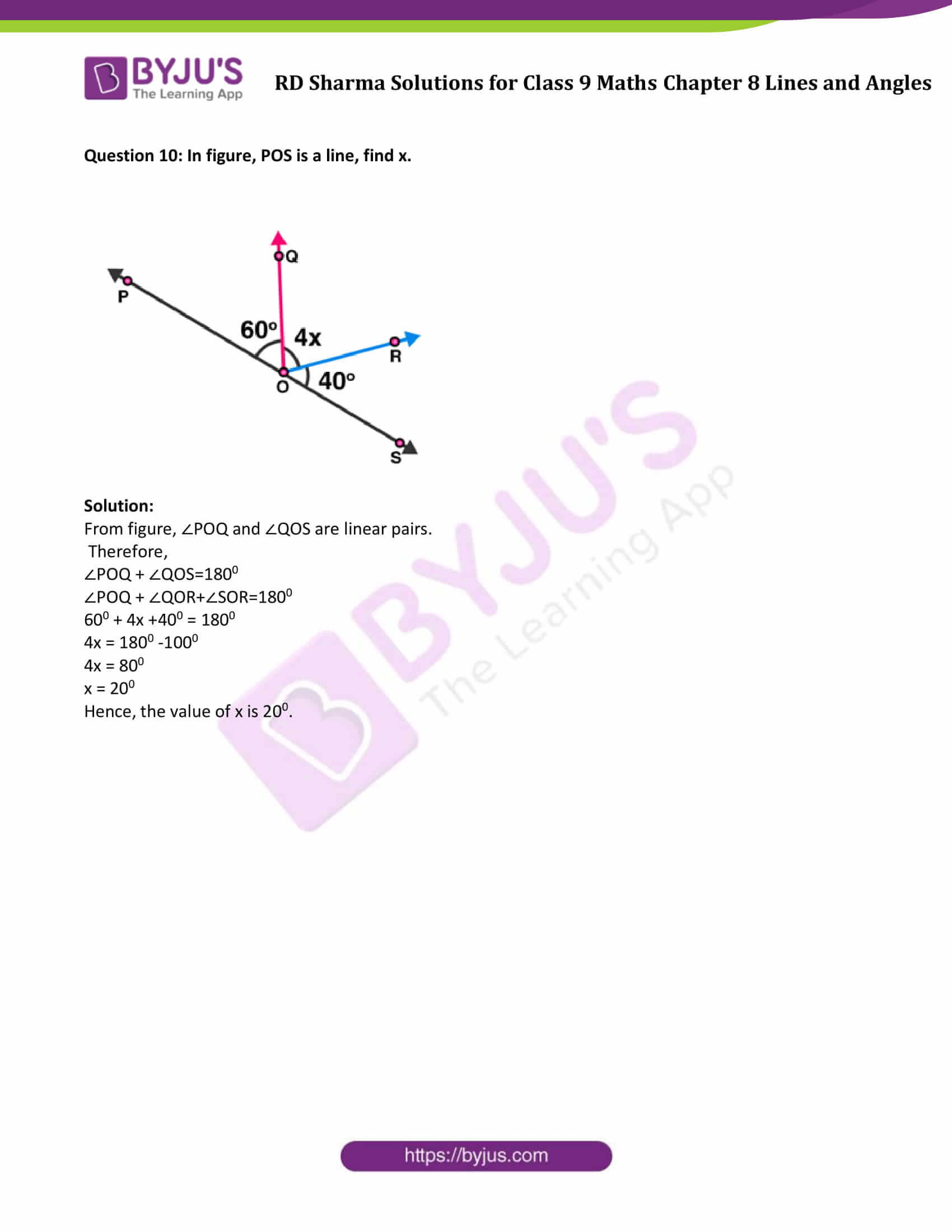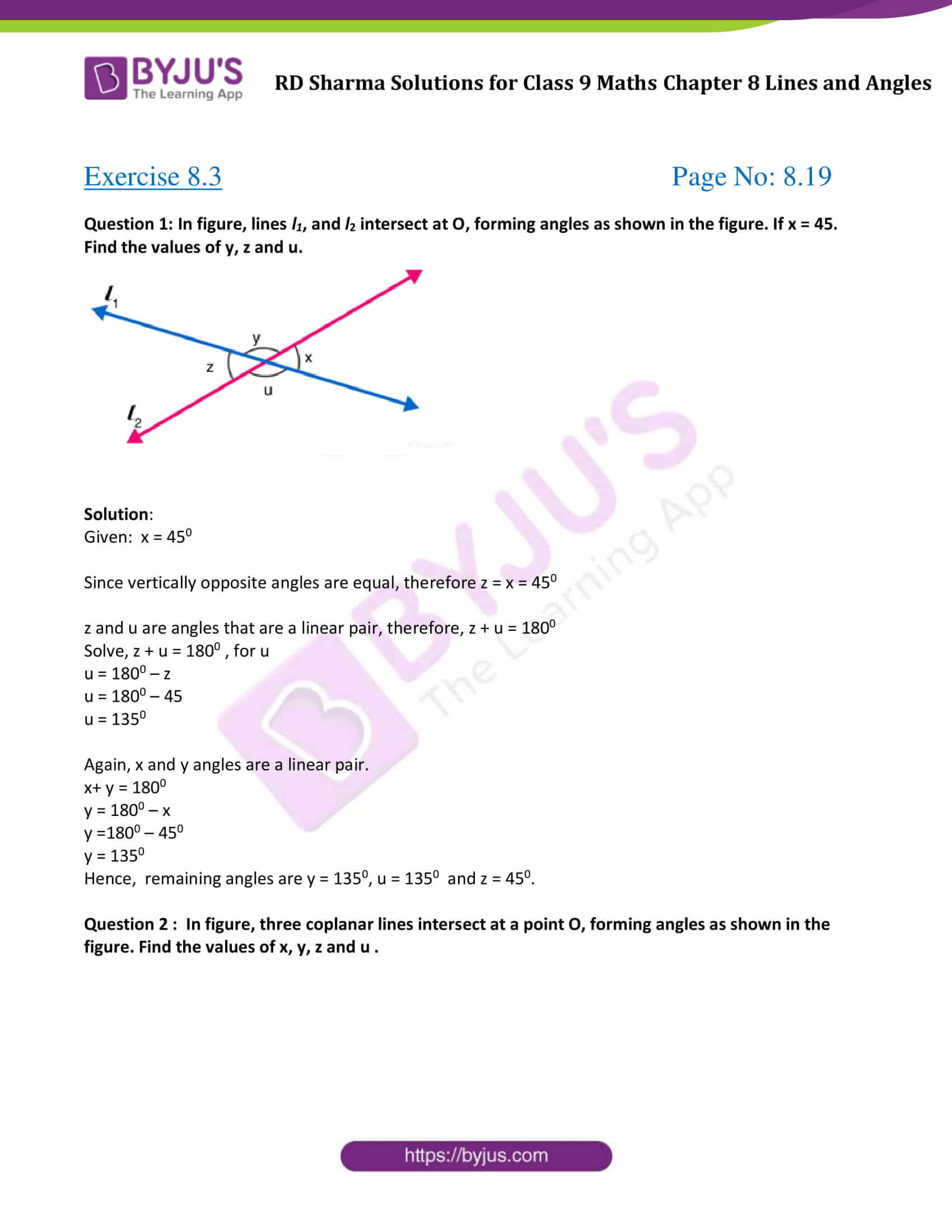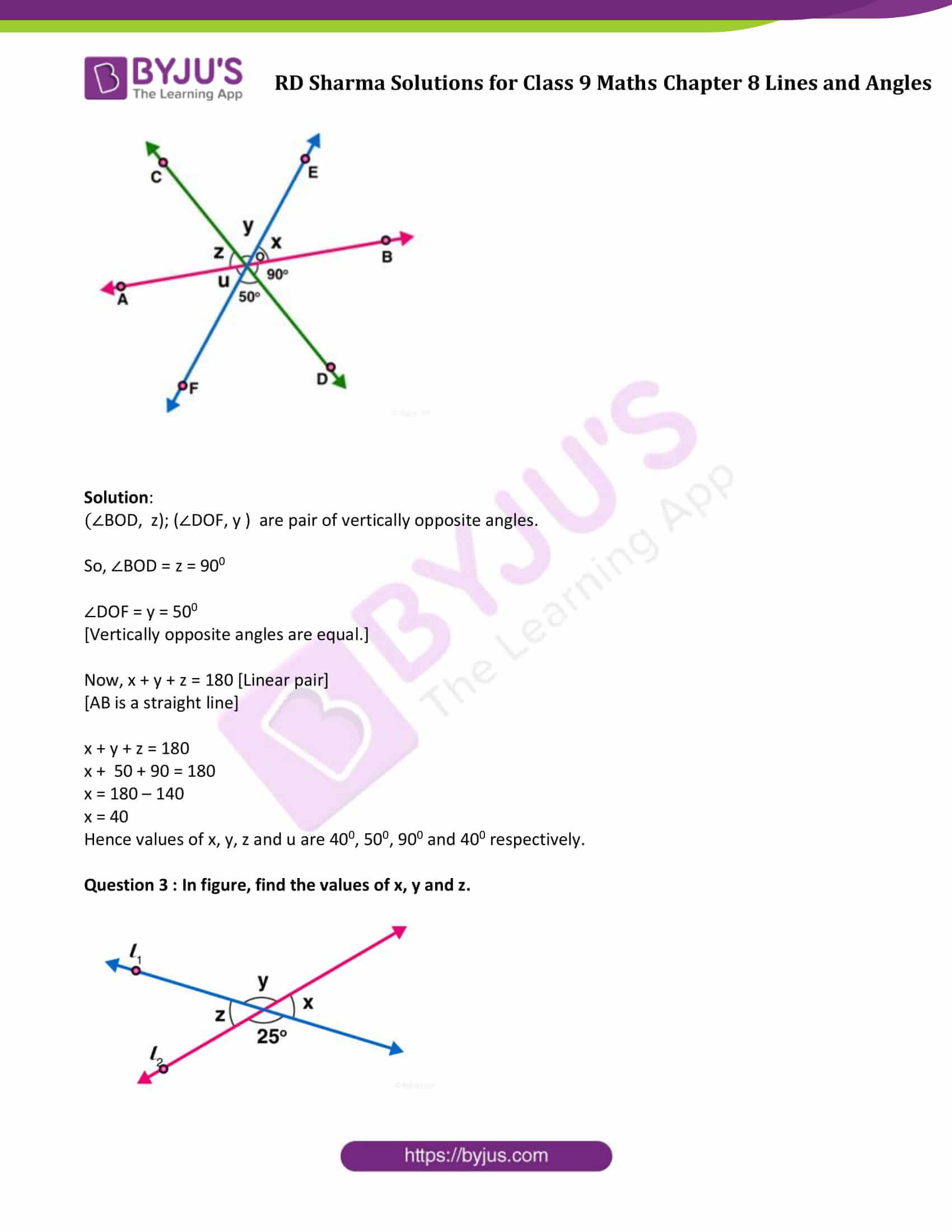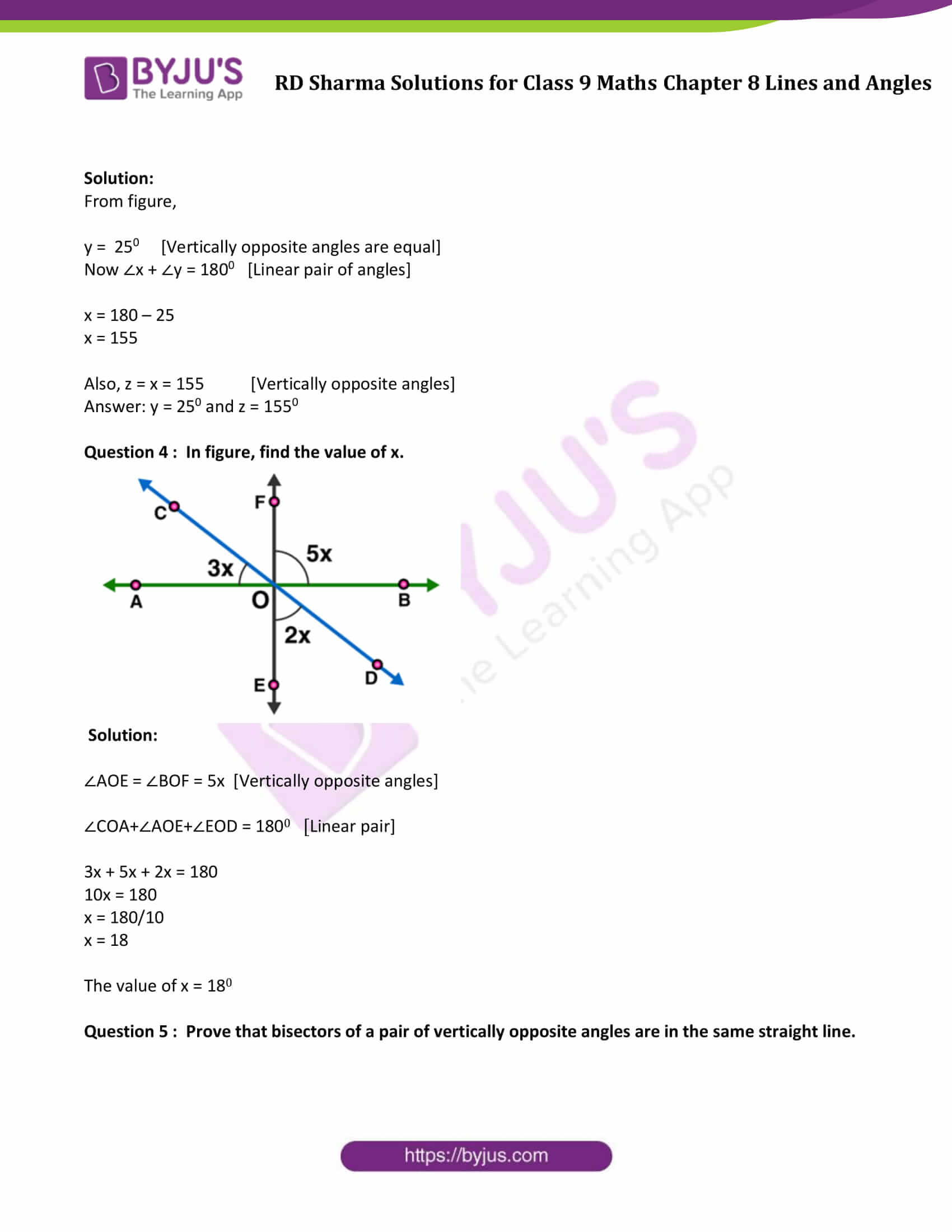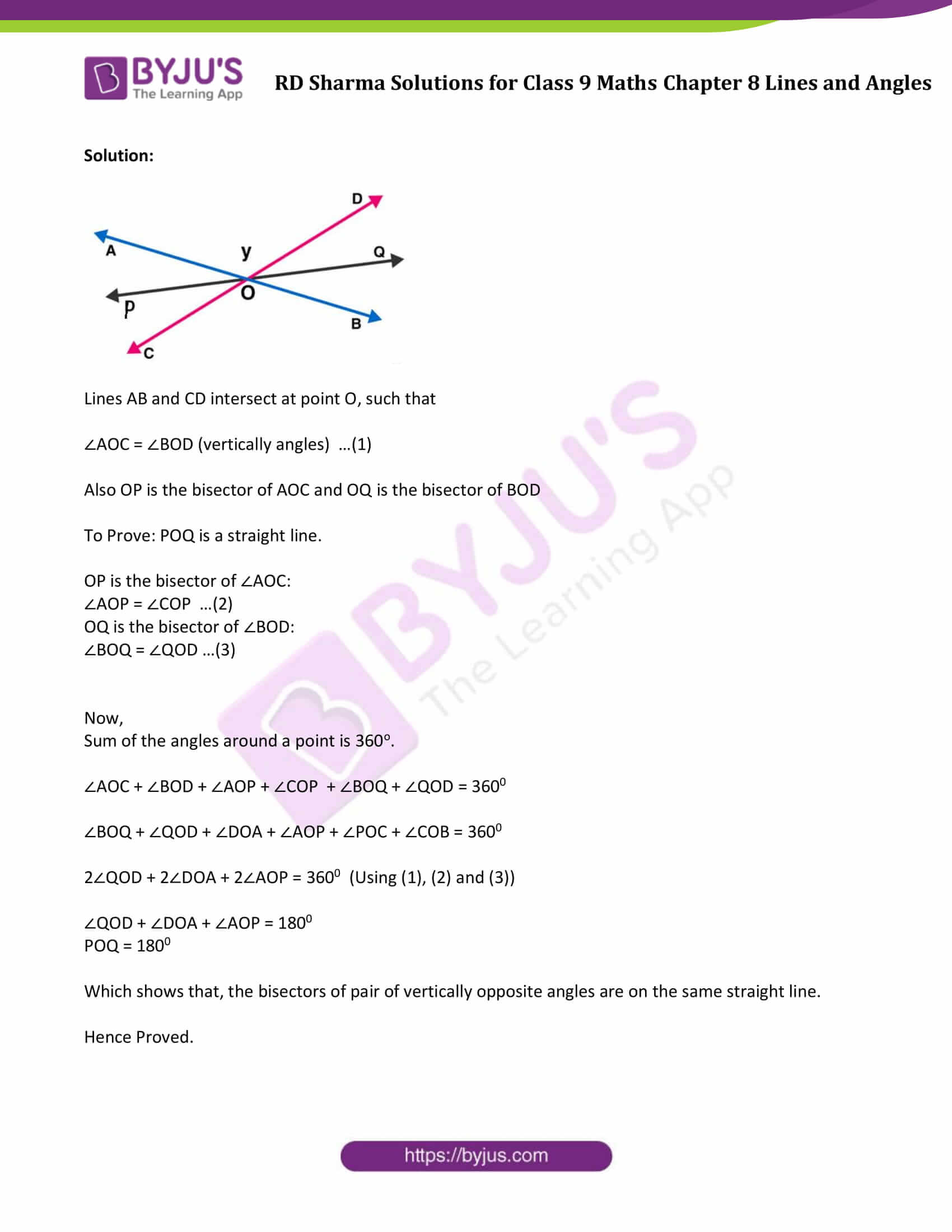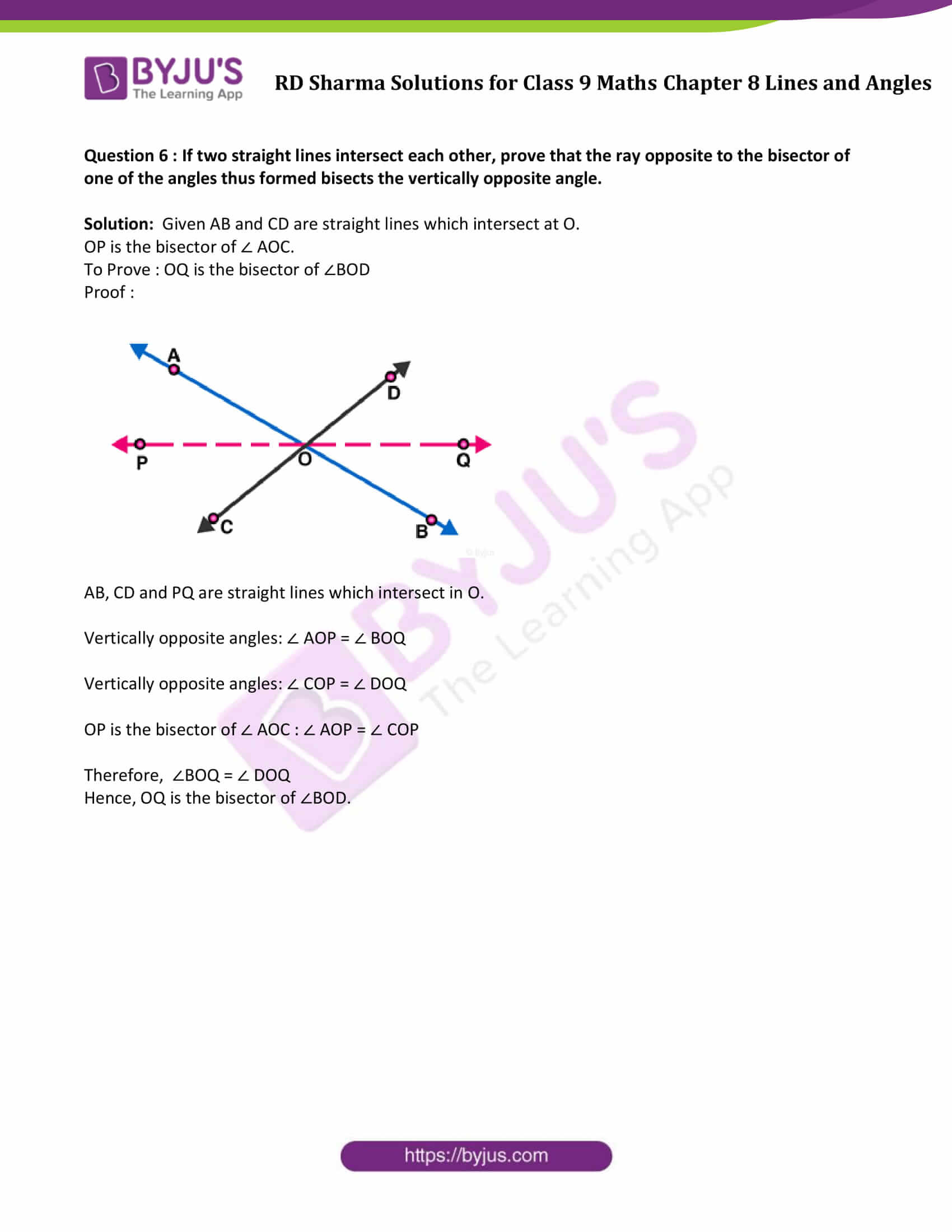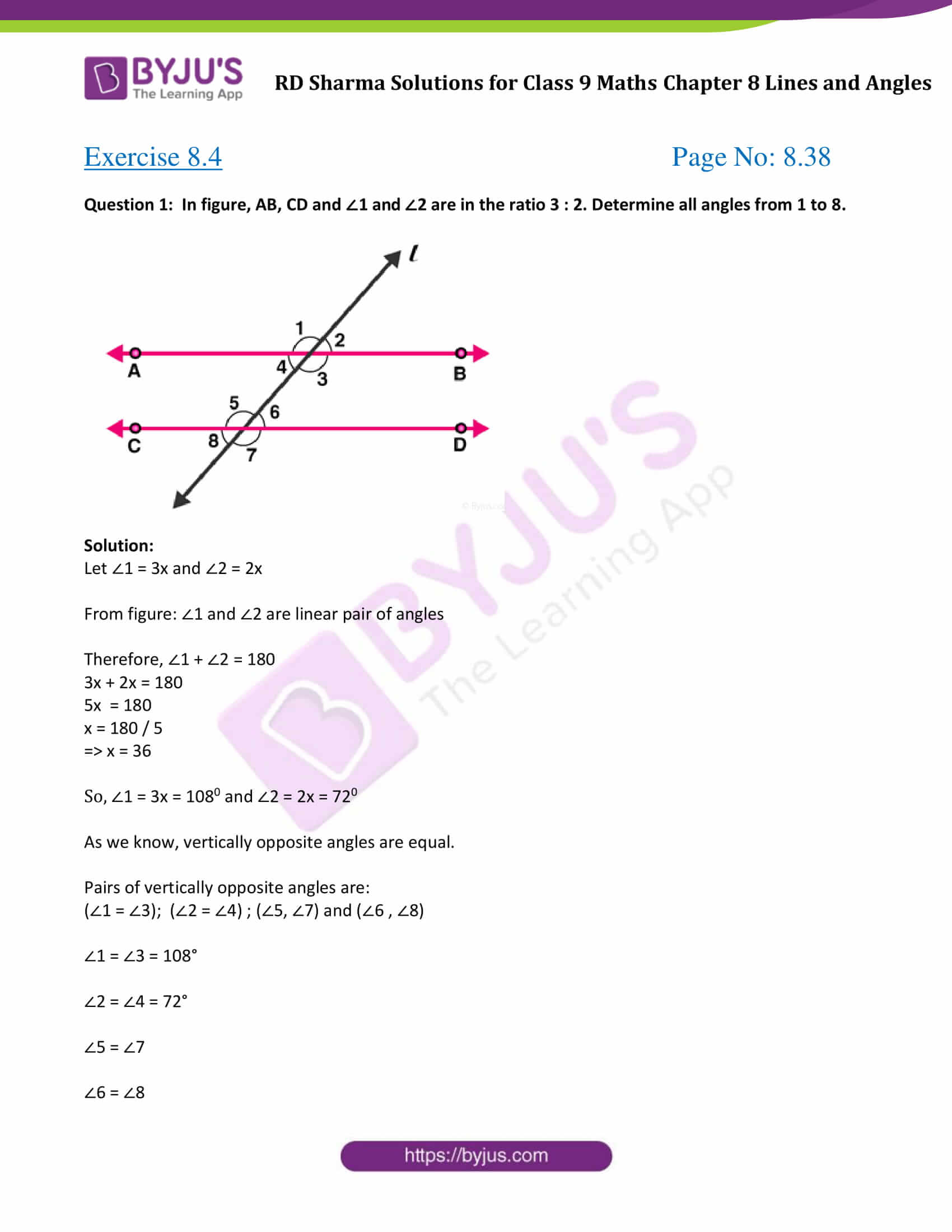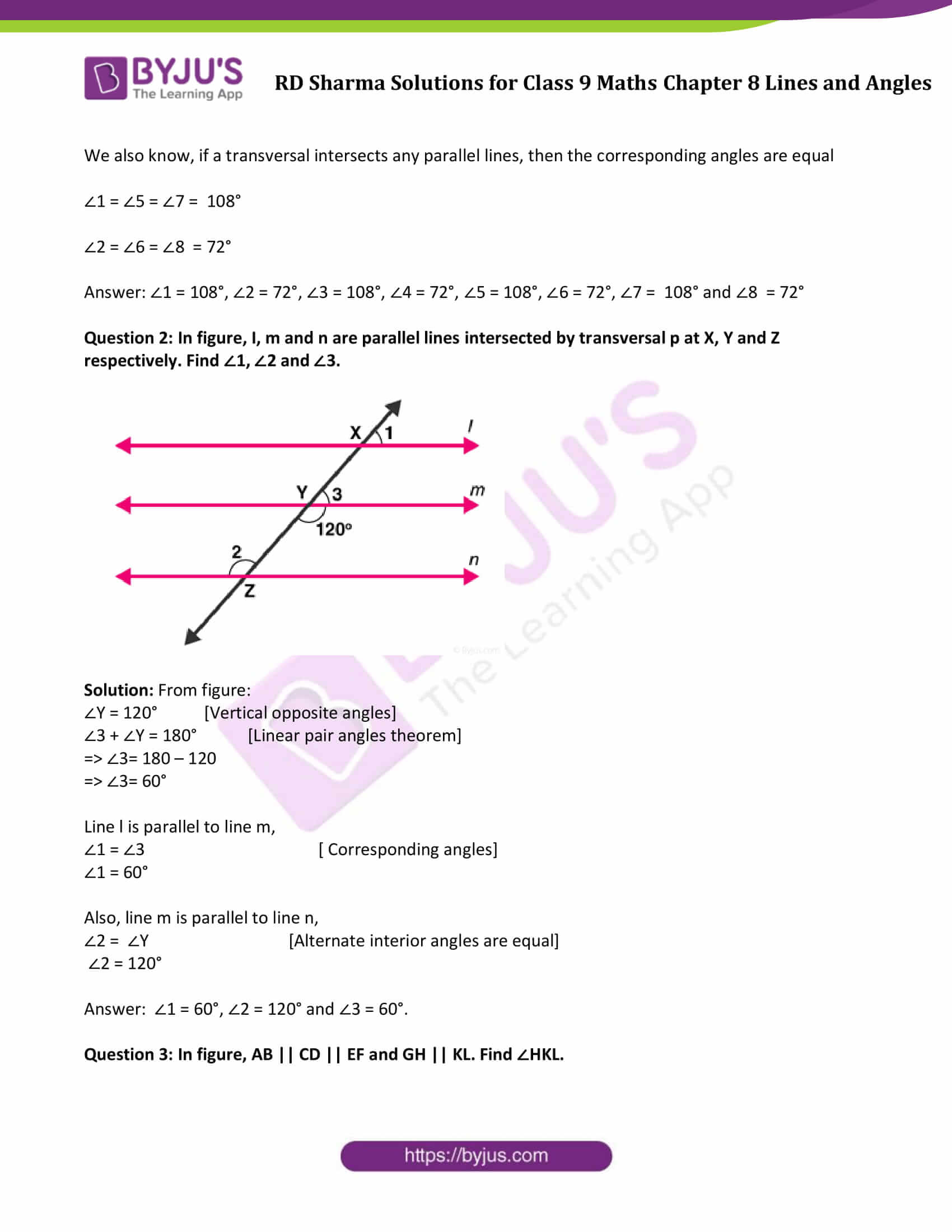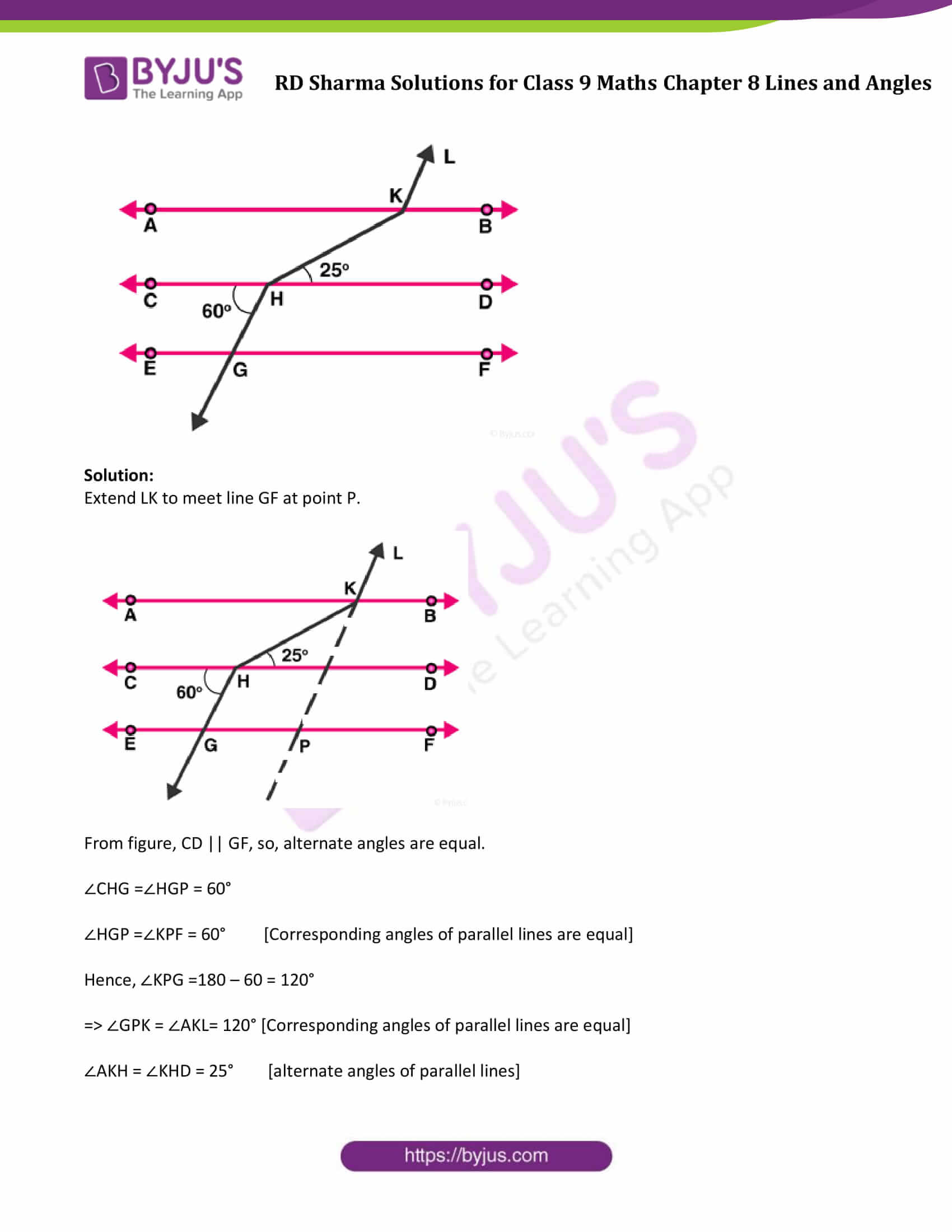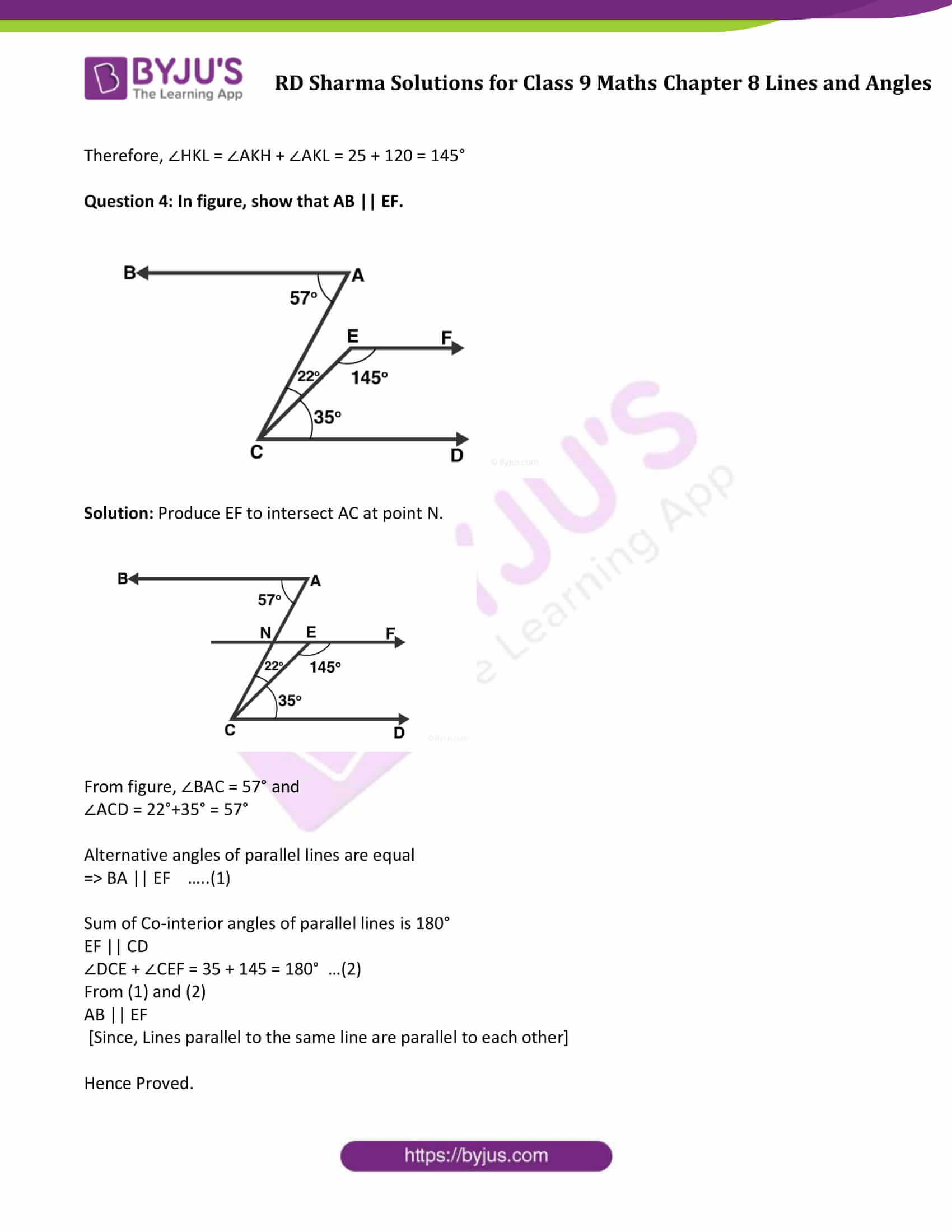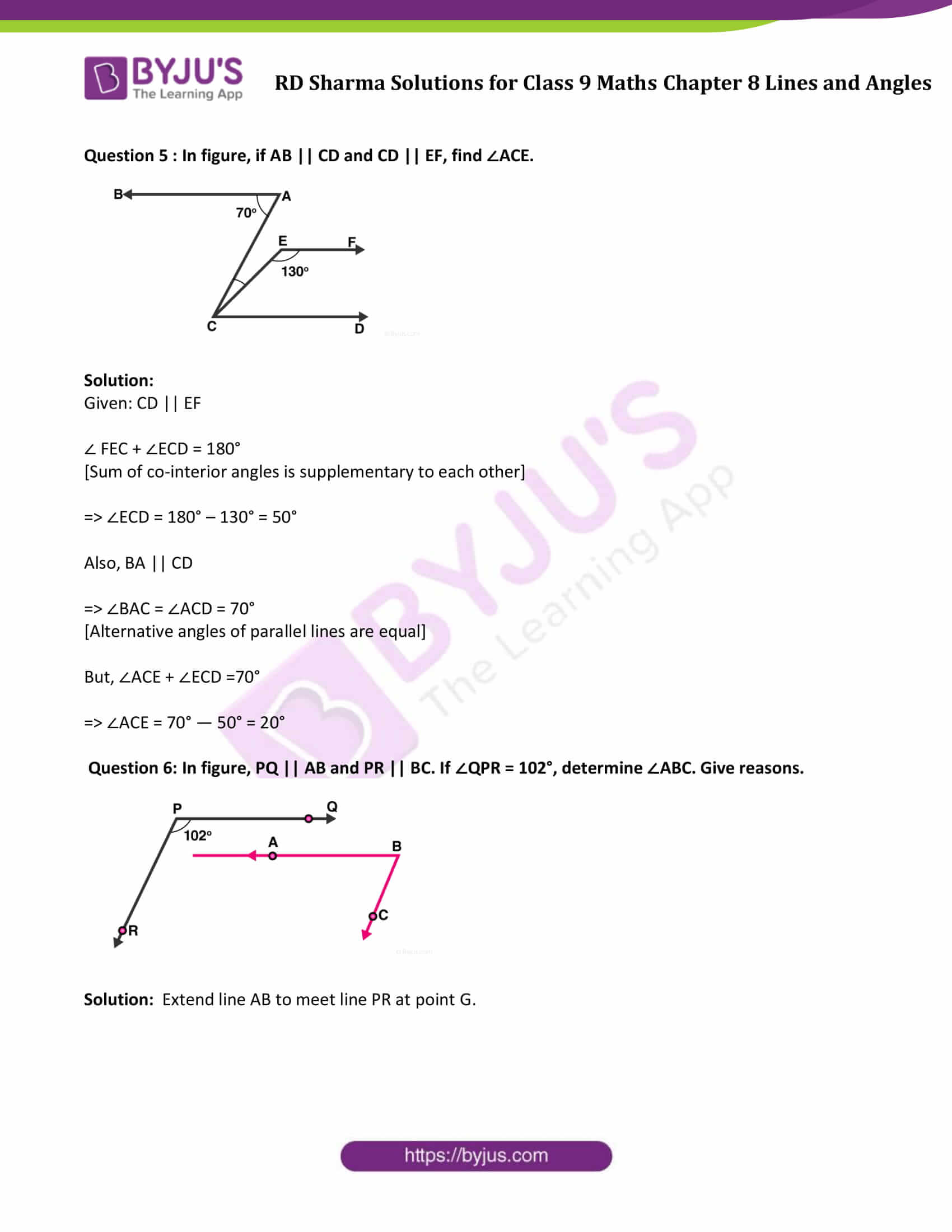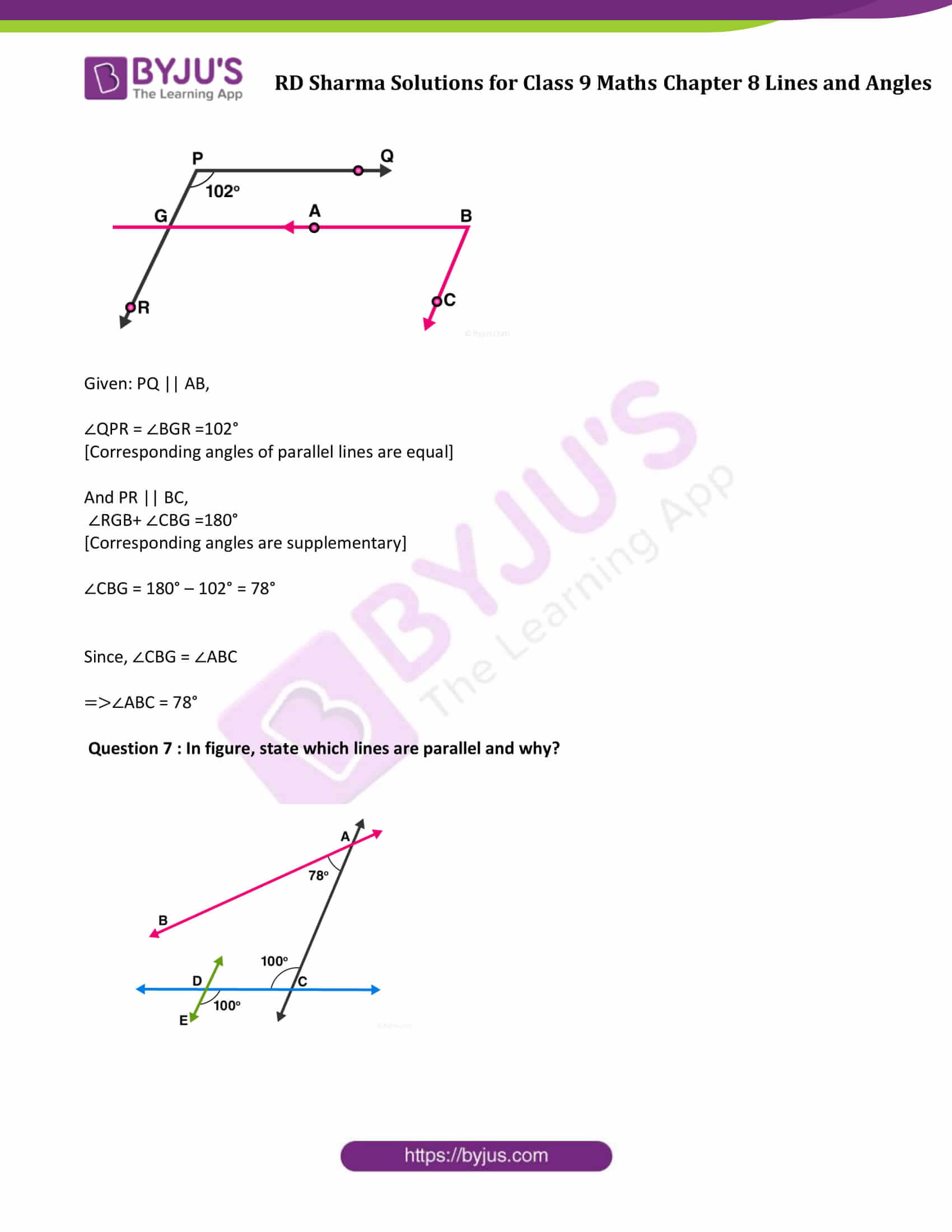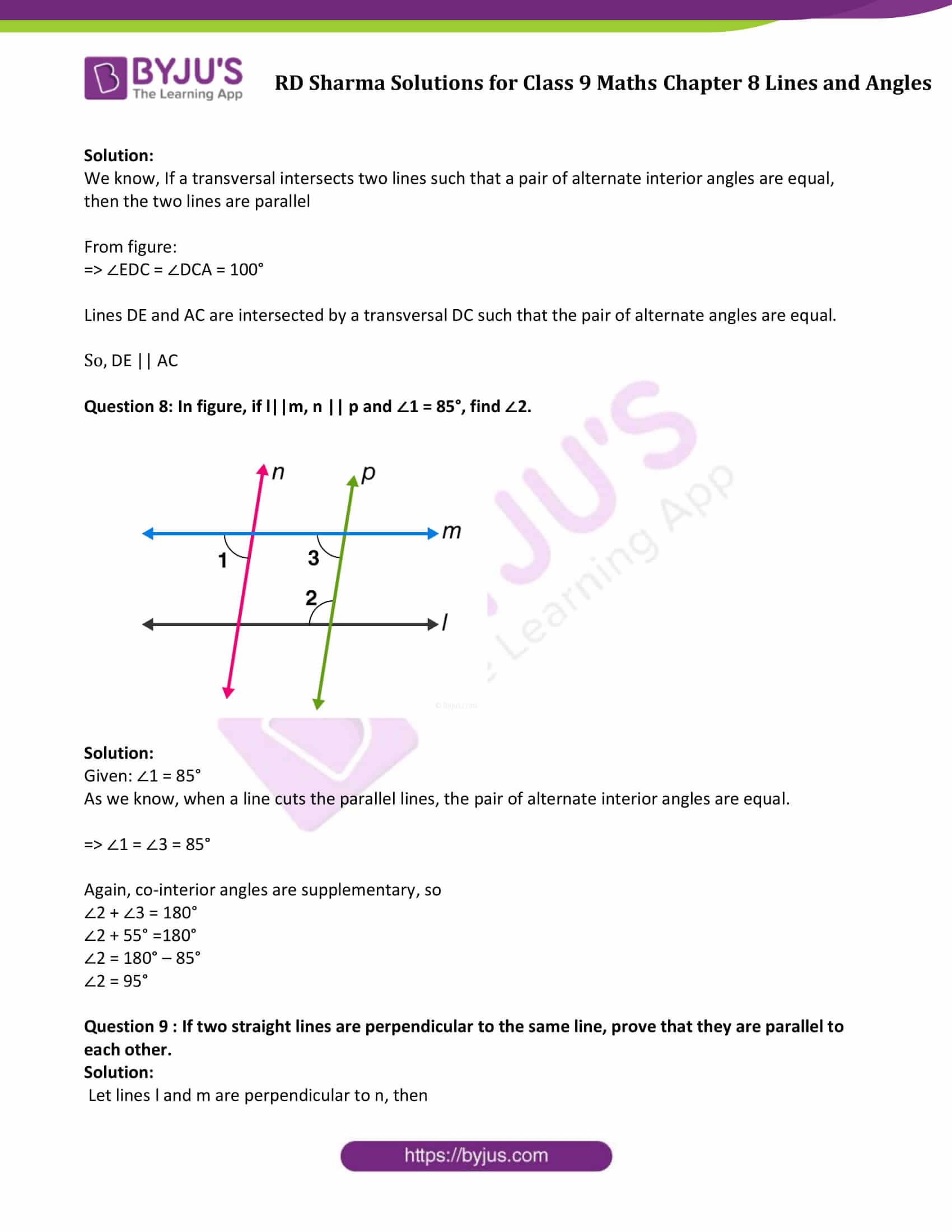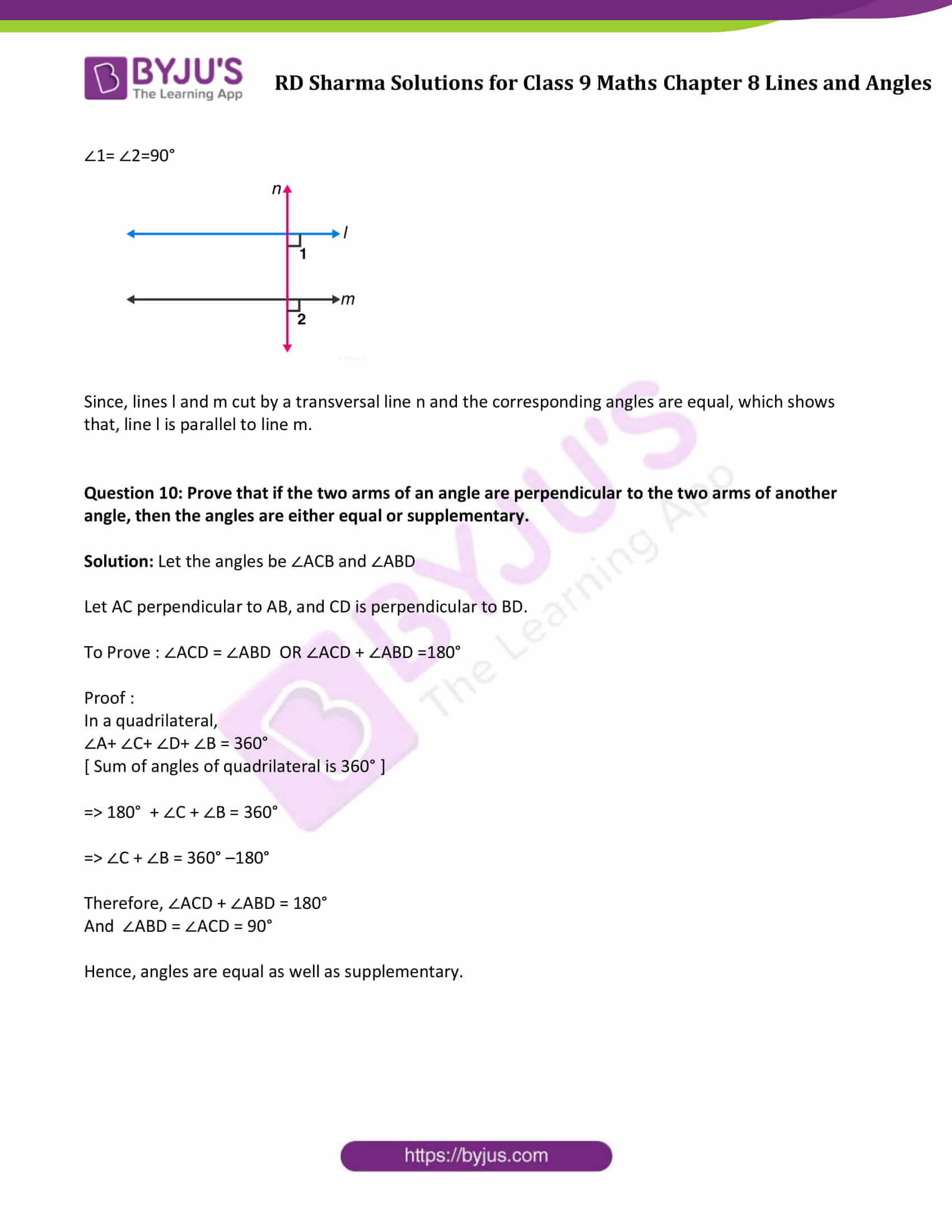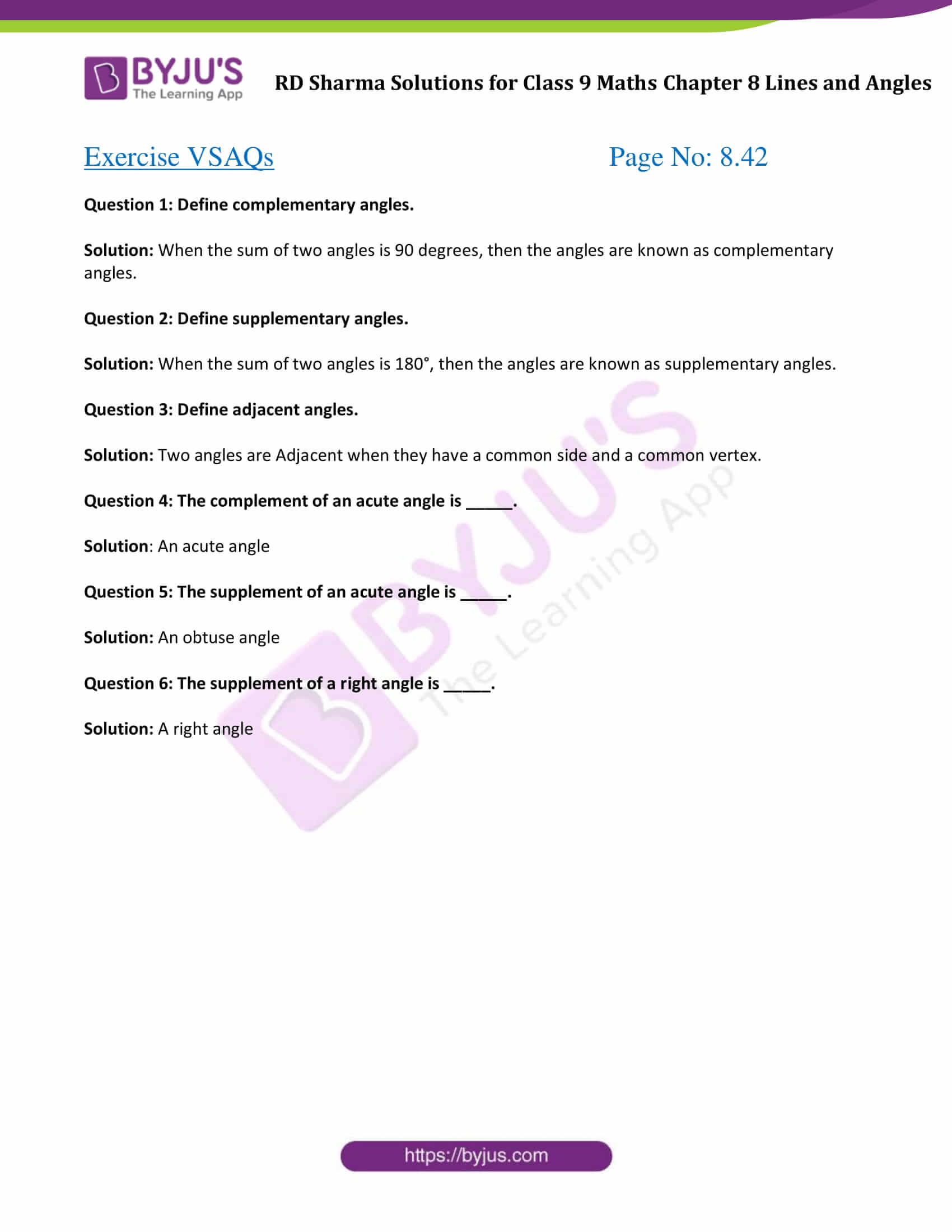### Access Answers to Maths RD Sharma Solutions for Class 9 Chapter 8 Lines and Angles

Exercise 8.1

Question 1: Write the complement of each of the following angles:

(i)200

(ii)350

(iii)900

(iv) 770

(v)300

Solution:

(i) The sum of an angle and its complement = 900

Therefore, the complement of 200 = 900 – 200 = 700

(ii) The sum of an angle and its complement = 900

Therefore, the complement of 35° = 90° – 35° = 55

(iii) The sum of an angle and its complement = 900

Therefore, the complement of 900 = 900 – 900 = 00

(iv) The sum of an angle and its complement = 900

Therefore, the complement of 770 = 90° – 770 = 130

(v) The sum of an angle and its complement = 900

Therefore, the complement of 300 = 900 – 300 = 600

Question 2 : Write the supplement of each of the following angles:

(i) 540

(ii) 1320

(iii) 1380

Solution:

(i) The sum of an angle and its supplement = 1800.

Therefore supplement of angle 540 = 1800 – 540 = 1260

(ii) The sum of an angle and its supplement = 1800.

Therefore supplement of angle 1320 = 1800 – 1320 = 480

(iii) The sum of an angle and its supplement = 1800.

Therefore supplement of angle 1380 = 1800 – 1380 = 420

Question 3: If an angle is 280 less than its complement, find its measure?

Solution:

Let the measure of any angle is ‘ a ‘ degrees

Thus, its complement will be (90 – a) 0

So, the required angle = Complement of a – 28

a = ( 90 – a ) – 28

2a = 62

a = 31

Hence, the angle measured is 310.

Question 4 : If an angle is 30° more than one half of its complement, find the measure of the angle?

Solution:

Let an angle measured by ‘ a ‘ in degrees

Thus, its complement will be (90 – a) 0

Required Angle = 300 + complement/2

a = 300 + ( 90 – a ) 0 / 2

a + a/2 = 300 + 450

3a/2 = 750

a = 500

Therefore, the measure of required angle is 500.

Question 5 : Two supplementary angles are in the ratio 4:5. Find the angles?

Solution:

Two supplementary angles are in the ratio 4:5.

Let us say, the angles are 4a and 5a (in degrees)

Since angle are supplementary angles;

Which implies, 4a + 5a = 1800

9a = 1800

a = 200

Therefore, 4a = 4 (20) = 800 and

5(a) = 5 (20) = 1000

Hence, required angles are 80° and 1000.

Question 6 : Two supplementary angles differ by 480. Find the angles?

Solution: Given: Two supplementary angles differ by 480.

Consider a0 be one angle then its supplementary angle will be equal to (180 – a) 0

According to the question;

(180 – a ) – x = 48

(180 – 48 ) = 2a

132 = 2a

132/2 = a

Or a = 660

Therefore, 180 – a = 1140

Hence, the two angles are 660 and 1140.

Question 7: An angle is equal to 8 times its complement. Determine its measure?

Solution: Given: Required angle = 8 times of its complement

Consider a0 be one angle then its complementary angle will be equal to (90 – a) 0

According to the question;

a = 8 times of its complement

a = 8 ( 90 – a )

a = 720 – 8a

a + 8a = 720

9a = 720

a = 80

Therefore, the required angle is 800.

Exercise 8.2

Question 1: In the below Fig. OA and OB are opposite rays:

(i) If x = 250, what is the value of y?

(ii) If y = 350, what is the value of x?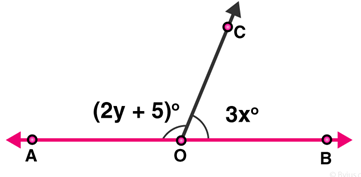Solution:

(i) Given: x = 25

From figure: ∠AOC and ∠BOC form a linear pair

Which implies, ∠AOC + ∠BOC = 1800

From the figure, ∠AOC = 2y + 5 and ∠BOC = 3x

∠AOC + ∠BOC = 1800

(2y + 5) + 3x = 180

(2y + 5) + 3 (25) = 180

2y + 5 + 75 = 180

2y + 80 = 180

2y = 100

y = 100/2 = 50

Therefore, y = 500

(ii) Given: y = 350

From figure: ∠AOC + ∠BOC = 180° (Linear pair angles)

(2y + 5) + 3x = 180

(2(35) + 5) + 3x = 180

75 + 3x = 180

3x = 105

x = 35

Therefore, x = 350

Question 2: In the below figure, write all pairs of adjacent angles and all the linear pairs.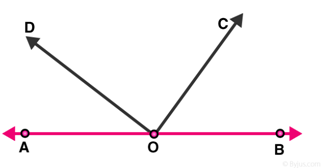Solution: From figure, pairs of adjacent angles are :

(∠AOC, ∠COB) ; (∠AOD, ∠BOD) ; (∠AOD, ∠COD) ; (∠BOC, ∠COD)

And Linear pair of angles are (∠AOD, ∠BOD) and (∠AOC, ∠BOC).

[As ∠AOD + ∠BOD = 1800 and ∠AOC+ ∠BOC = 1800.]

Question 3 : In the given figure, find x. Further find ∠BOC , ∠COD and ∠AOD.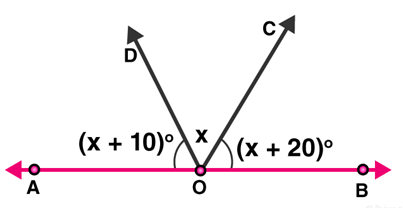Solution:

From figure, ∠AOD and ∠BOD form a linear pair,

Therefore, ∠AOD+ ∠BOD = 1800

Also, ∠AOD + ∠BOC + ∠COD = 1800

Given: ∠AOD = (x+10) 0 , ∠COD = x0 and ∠BOC = (x + 20) 0

( x + 10 ) + x + ( x + 20 ) = 180

3x + 30 = 180

3x = 180 – 30

x = 150/3

x = 500

Now,

∠AOD=(x+10) =50 + 10 = 60

∠COD = x = 50

∠BOC = (x+20) = 50 + 20 = 70

Hence, ∠AOD=600, ∠COD=500 and ∠BOC=700

Question 4: In figure, rays OA, OB, OC, OD and OE have the common end point 0. Show that ∠AOB+∠BOC+∠COD+∠DOE+∠EOA=360°.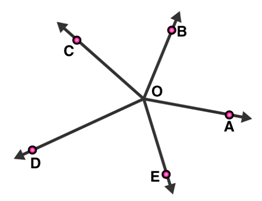Solution:

Given: Rays OA, OB, OC, OD and OE have the common endpoint O.

Draw an opposite ray OX to ray OA, which make a straight line AX.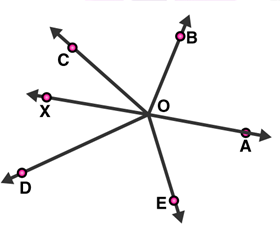From figure:

∠AOB and ∠BOX are linear pair angles, therefore,

∠AOB +∠BOX = 1800

Or, ∠AOB + ∠BOC + ∠COX = 1800 —–—–(1)

Also,

∠AOE and ∠EOX are linear pair angles, therefore,

∠AOE+∠EOX =180°

Or, ∠AOE + ∠DOE + ∠DOX = 1800 —–(2)

By adding equations, (1) and (2), we get;

∠AOB + ∠BOC + ∠COF + ∠AOE + ∠DOE + ∠DOX = 1800 + 1800

∠AOB + ∠BOC + ∠COD + ∠DOE + ∠EOA = 3600

Hence Proved.

Question 5 : In figure, ∠AOC and ∠BOC form a linear pair. If a – 2b = 30°, find a and b?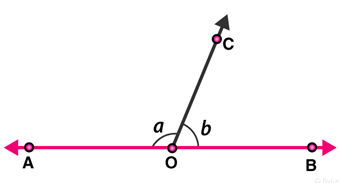Solution:

Given : ∠AOC and ∠BOC form a linear pair.

=> a + b = 1800 …..(1)

a – 2b = 300 …(2) (given)

On subtracting equation (2) from (1), we get

a + b – a + 2b = 180 – 30

3b = 150

b = 150/3

b = 500

Since, a – 2b = 300

a – 2(50) = 30

a = 30 + 100

a = 1300

Therefore, the values of a and b are 130° and 50° respectively.

Question 6: How many pairs of adjacent angles are formed when two lines intersect at a point?

Solution: Four pairs of adjacent angles are formed when two lines intersect each other at a single point.

For example, Let two lines AB and CD intersect at point O.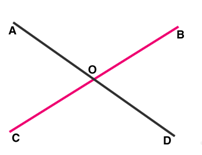The 4 pair of adjacent angles are :

(∠AOD,∠DOB),(∠DOB,∠BOC),(∠COA, ∠AOD) and (∠BOC,∠COA).

Question 7: How many pairs of adjacent angles, in all, can you name in figure given?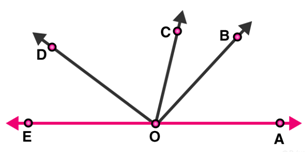Solution: Number of Pairs of adjacent angles, from the figure, are :

∠EOC and ∠DOC

∠EOD and ∠DOB

∠DOC and ∠COB

∠EOD and ∠DOA

∠DOC and ∠COA

∠BOC and ∠BOA

∠BOA and ∠BOD

∠BOA and ∠BOE

∠EOC and ∠COA

∠EOC and ∠COB

Hence, there are 10 pairs of adjacent angles.

Question 8: In figure, determine the value of x.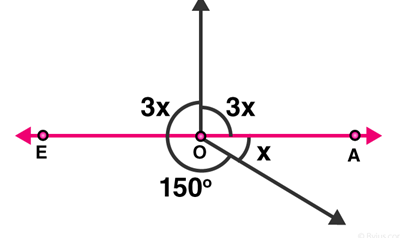Solution:

The sum of all the angles around a point O is equal to 360°.

Therefore,

3x + 3x + 150 + x = 3600

7x = 3600 – 1500

7x = 2100

x = 210/7

x = 300

Hence, the value of x is 30°.

Question 9: In figure, AOC is a line, find x.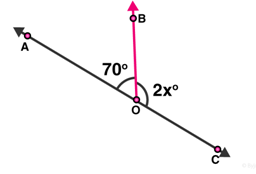Solution:

From the figure, ∠AOB and ∠BOC are linear pairs,

∠AOB +∠BOC =180°

70 + 2x = 180

2x = 180 – 70

2x = 110

x = 110/2

x = 55

Therefore, the value of x is 550.

Question 10: In figure, POS is a line, find x.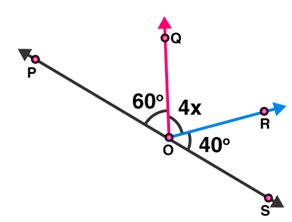Solution:

From figure, ∠POQ and ∠QOS are linear pairs.

Therefore,

∠POQ + ∠QOS=1800

∠POQ + ∠QOR+∠SOR=1800

600 + 4x +400 = 1800

4x = 1800 -1000

4x = 800

x = 200

Hence, the value of x is 200.

Exercise 8.3

Question 1: In figure, lines l1, and l2 intersect at O, forming angles as shown in the figure. If x = 45. Find the values of y, z and u.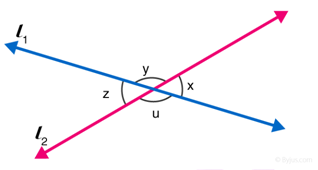Solution:

Given: x = 450

Since vertically opposite angles are equal, therefore z = x = 450

z and u are angles that are a linear pair, therefore, z + u = 1800

Solve, z + u = 1800 , for u

u = 1800 – z

u = 1800 – 45

u = 1350

Again, x and y angles are a linear pair.

x+ y = 1800

y = 1800 – x

y =1800 – 450

y = 1350

Hence, remaining angles are y = 1350, u = 1350 and z = 450.

Question 2 : In figure, three coplanar lines intersect at a point O, forming angles as shown in the figure. Find the values of x, y, z and u .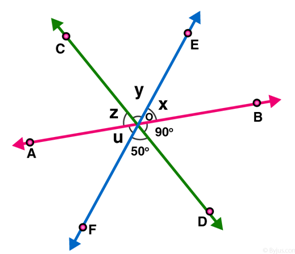Solution:

(∠BOD, z); (∠DOF, y ) are pair of vertically opposite angles.

So, ∠BOD = z = 900

∠DOF = y = 500

[Vertically opposite angles are equal.]

Now, x + y + z = 180 [Linear pair] [AB is a straight line]

x + y + z = 180

x + 50 + 90 = 180

x = 180 – 140

x = 40

Hence values of x, y, z and u are 400, 500, 900 and 400 respectively.

Question 3 : In figure, find the values of x, y and z.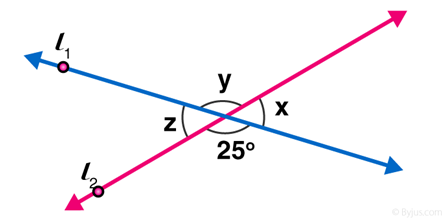Solution:

From figure,

y = 250 [Vertically opposite angles are equal]

Now ∠x + ∠y = 1800 [Linear pair of angles]

x = 180 – 25

x = 155

Also, z = x = 155 [Vertically opposite angles]

Answer: y = 250 and z = 1550

Question 4 : In figure, find the value of x.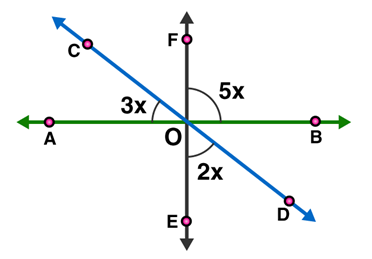Solution:

∠AOE = ∠BOF = 5x [Vertically opposite angles]

∠COA+∠AOE+∠EOD = 1800 [Linear pair]

3x + 5x + 2x = 180

10x = 180

x = 180/10

x = 18

The value of x = 180

Question 5 : Prove that bisectors of a pair of vertically opposite angles are in the same straight line.

Solution: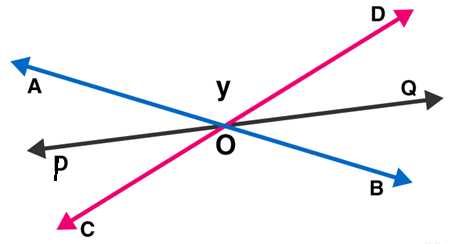Lines AB and CD intersect at point O, such that

∠AOC = ∠BOD (vertically angles) …(1)

Also OP is the bisector of AOC and OQ is the bisector of BOD

To Prove: POQ is a straight line.

OP is the bisector of ∠AOC:

∠AOP = ∠COP …(2)

OQ is the bisector of ∠BOD:

∠BOQ = ∠QOD …(3)

Now,

Sum of the angles around a point is 360o.

∠AOC + ∠BOD + ∠AOP + ∠COP + ∠BOQ + ∠QOD = 3600

∠BOQ + ∠QOD + ∠DOA + ∠AOP + ∠POC + ∠COB = 3600

2∠QOD + 2∠DOA + 2∠AOP = 3600 (Using (1), (2) and (3))

∠QOD + ∠DOA + ∠AOP = 1800

POQ = 1800

Which shows that, the bisectors of pair of vertically opposite angles are on the same straight line.

Hence Proved.

Question 6 : If two straight lines intersect each other, prove that the ray opposite to the bisector of one of the angles thus formed bisects the vertically opposite angle.

Solution: Given AB and CD are straight lines which intersect at O.

OP is the bisector of ∠ AOC.

To Prove : OQ is the bisector of ∠BOD

Proof :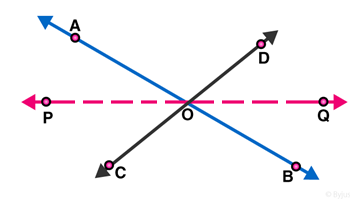AB, CD and PQ are straight lines which intersect in O.

Vertically opposite angles: ∠ AOP = ∠ BOQ

Vertically opposite angles: ∠ COP = ∠ DOQ

OP is the bisector of ∠ AOC : ∠ AOP = ∠ COP

Therefore,  ∠BOQ = ∠ DOQ

Hence, OQ is the bisector of ∠BOD.

Exercise 8.4

Question 1: In figure, AB, CD and ∠1 and ∠2 are in the ratio 3 : 2. Determine all angles from 1 to 8.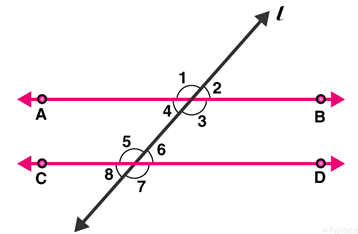Solution:

Let ∠1 = 3x and ∠2 = 2x

From figure: ∠1 and ∠2 are linear pair of angles

Therefore, ∠1 + ∠2 = 180

3x + 2x = 180

5x = 180

x = 180 / 5

=> x = 36

So, ∠1 = 3x = 1080 and ∠2 = 2x = 720

As we know, vertically opposite angles are equal.

Pairs of vertically opposite angles are:

(∠1 = ∠3); (∠2 = ∠4) ; (∠5, ∠7) and (∠6 , ∠8)

∠1 = ∠3 = 108°

∠2 = ∠4 = 72°

∠5 = ∠7

∠6 = ∠8

We also know, if a transversal intersects any parallel lines, then the corresponding angles are equal

∠1 = ∠5 = ∠7 = 108°

∠2 = ∠6 = ∠8 = 72°

Answer: ∠1 = 108°, ∠2 = 72°, ∠3 = 108°, ∠4 = 72°, ∠5 = 108°, ∠6 = 72°, ∠7 = 108° and ∠8 = 72°

Question 2: In figure, I, m and n are parallel lines intersected by transversal p at X, Y and Z respectively. Find ∠1, ∠2 and ∠3.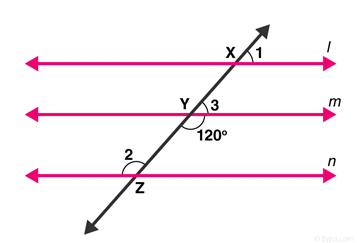Solution: From figure:

∠Y = 120° [Vertical opposite angles]

∠3 + ∠Y = 180° [Linear pair angles theorem]

=> ∠3= 180 – 120

=> ∠3= 60°

Line l is parallel to line m,

∠1 = ∠3 [ Corresponding angles]

∠1 = 60°

Also, line m is parallel to line n,

∠2 = ∠Y [Alternate interior angles are equal]

∠2 = 120°

Answer: ∠1 = 60°, ∠2 = 120° and ∠3 = 60°.

Question 3: In figure, AB || CD || EF and GH || KL. Find ∠HKL.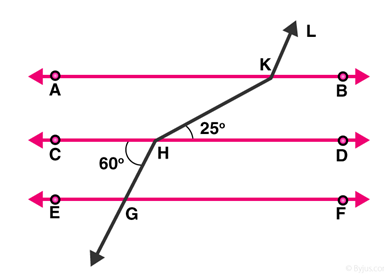Solution:

Extend LK to meet line GF at point P.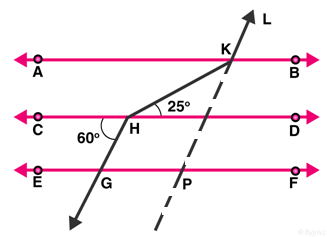From figure, CD || GF, so, alternate angles are equal.

∠CHG =∠HGP = 60°

∠HGP =∠KPF = 60° [Corresponding angles of parallel lines are equal]

Hence, ∠KPG =180 – 60 = 120°

=> ∠GPK = ∠AKL= 120° [Corresponding angles of parallel lines are equal]

∠AKH = ∠KHD = 25° [alternate angles of parallel lines]

Therefore, ∠HKL = ∠AKH + ∠AKL = 25 + 120 = 145°

Question 4: In figure, show that AB || EF.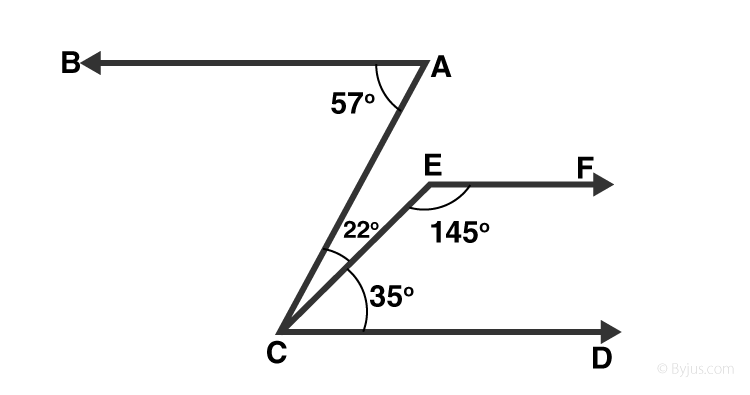Solution: Produce EF to intersect AC at point N.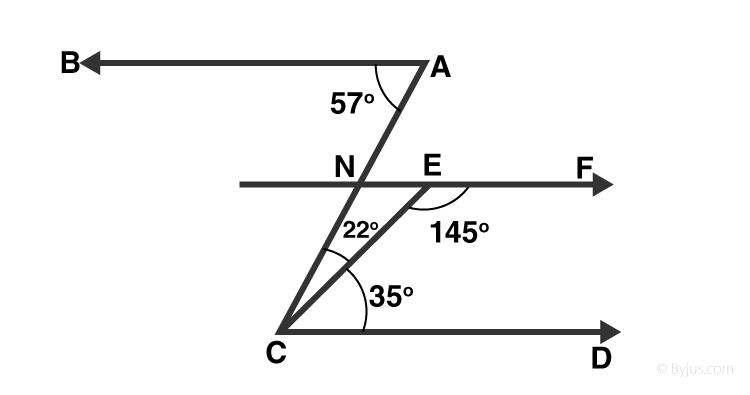From figure, ∠BAC = 57° and

∠ACD = 22°+35° = 57°

Alternative angles of parallel lines are equal

=> BA || EF …..(1)

Sum of Co-interior angles of parallel lines is 180°

EF || CD

∠DCE + ∠CEF = 35 + 145 = 180° …(2)

From (1) and (2)

AB || EF

[Since, Lines parallel to the same line are parallel to each other]

Hence Proved.

Question 5 : In figure, if AB || CD and CD || EF, find ∠ACE.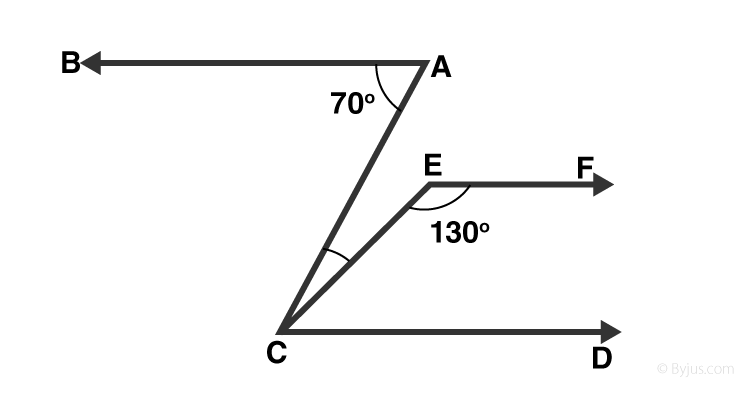Solution:

Given: CD || EF

∠ FEC + ∠ECD = 180°

[Sum of co-interior angles is supplementary to each other]

=> ∠ECD = 180° – 130° = 50°

Also, BA || CD

=> ∠BAC = ∠ACD = 70°

[Alternative angles of parallel lines are equal]

But, ∠ACE + ∠ECD =70°

=> ∠ACE = 70° — 50° = 20°

Question 6: In figure, PQ || AB and PR || BC. If ∠QPR = 102°, determine ∠ABC. Give reasons.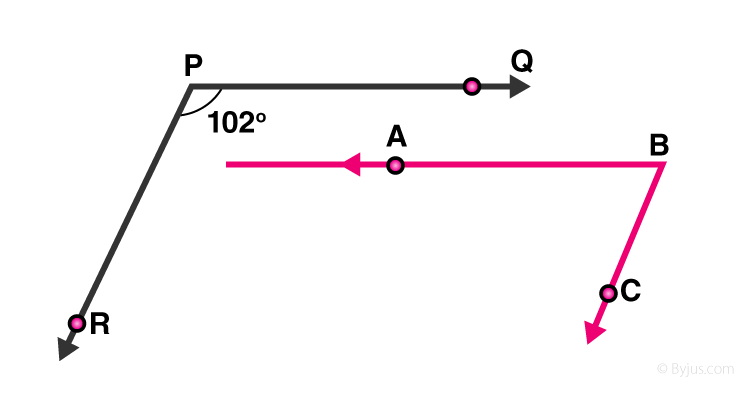Solution: Extend line AB to meet line PR at point G.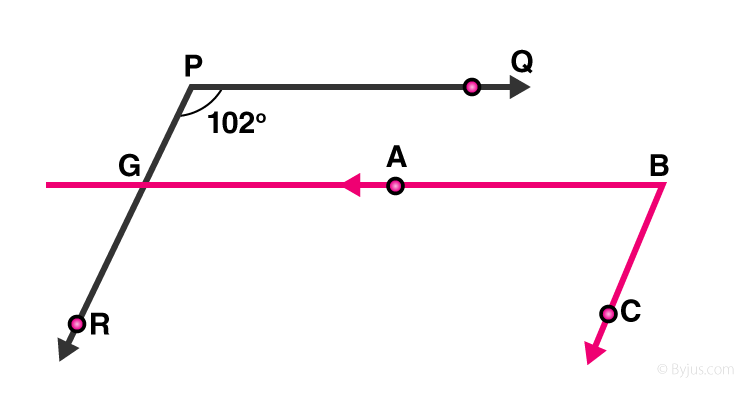Given: PQ || AB,

∠QPR = ∠BGR =102°

[Corresponding angles of parallel lines are equal]

And PR || BC,

∠RGB+ ∠CBG =180°

[Corresponding angles are supplementary]

∠CBG = 180° – 102° = 78°

Since, ∠CBG = ∠ABC

=>∠ABC = 78°

Question 7 : In figure, state which lines are parallel and why?Solution:

We know, If a transversal intersects two lines such that a pair of alternate interior angles are equal, then the two lines are parallel

From figure:

=> ∠EDC = ∠DCA = 100°

Lines DE and AC are intersected by a transversal DC such that the pair of alternate angles are equal.

So, DE || AC

Question 8: In figure, if l||m, n || p and ∠1 = 85°, find ∠2.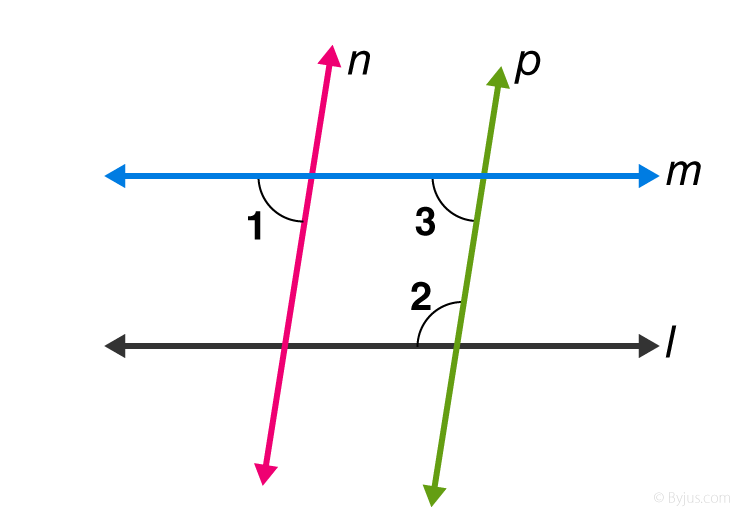Solution:

Given: ∠1 = 85°

As we know, when a line cuts the parallel lines, the pair of alternate interior angles are equal.

=> ∠1 = ∠3 = 85°

Again, co-interior angles are supplementary, so

∠2 + ∠3 = 180°

∠2 + 55° =180°

∠2 = 180° – 85°

∠2 = 95°

Question 9 : If two straight lines are perpendicular to the same line, prove that they are parallel to each other.

Solution:

Let lines l and m are perpendicular to n, then

∠1= ∠2=90°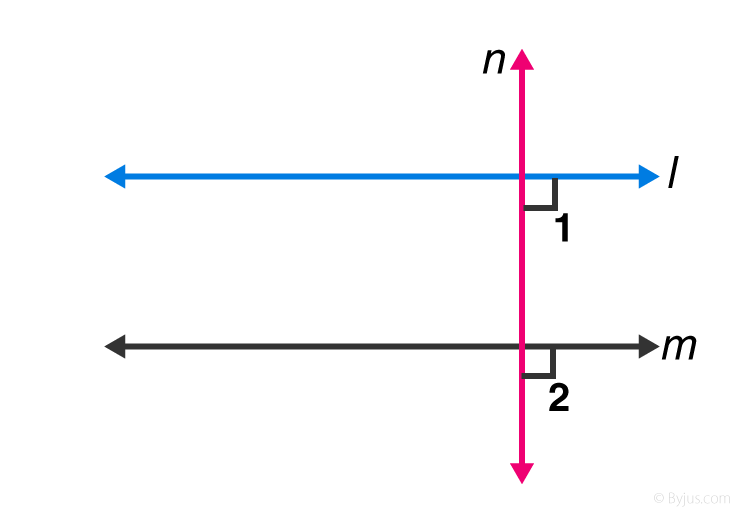Since, lines l and m cut by a transversal line n and the corresponding angles are equal, which shows that, line l is parallel to line m.

Question 10: Prove that if the two arms of an angle are perpendicular to the two arms of another angle, then the angles are either equal or supplementary.

Solution: Let the angles be ∠ACB and ∠ABD

Let AC perpendicular to AB, and CD is perpendicular to BD.

To Prove : ∠ACD = ∠ABD OR ∠ACD + ∠ABD =180°

Proof :

∠A+ ∠C+ ∠D+ ∠B = 360°

[ Sum of angles of quadrilateral is 360° ]

=> 180° + ∠C + ∠B = 360°

=> ∠C + ∠B = 360° –180°

Therefore, ∠ACD + ∠ABD = 180°

And ∠ABD = ∠ACD = 90°

Hence, angles are equal as well as supplementary.

Exercise VSAQs

Question 1: Define complementary angles.

Solution: When the sum of two angles is 90 degrees, then the angles are known as complementary angles.

Question 2: Define supplementary angles.

Solution: When the sum of two angles is 180°, then the angles are known as supplementary angles.

Solution: Two angles are Adjacent when they have a common side and a common vertex.

Question 4: The complement of an acute angle is _____.

Solution: An acute angle

Question 5: The supplement of an acute angle is _____.

Solution: An obtuse angle

Question 6: The supplement of a right angle is _____.

Solution: A right angle

## RD Sharma Solutions for Class 9 Maths Chapter 8 Lines and Angles

In the 8th Chapter of Class 9 RD Sharma Solutions, students will study important concepts listed below:

• Introduction to Lines and Angles
• Angles
• Angles Axioms and Theorems
• Some angle relations
• Linear pair of angles
• Vertically opposite angles
• Angles made by a transversal with two lines
• Alternate interior angles
• Consecutive interior angles

## Frequently Asked Questions on RD Sharma Solutions for Class 9 Maths Chapter 8

### Does RD Sharma Solutions for Class 9 Maths Chapter 8 Lines and Angles provide accurate answers to all the problems?

Yes, students can refer to RD Sharma Solutions for Class 9 Maths Chapter 8 for precise answers to each problem of the textbook. In order to improve conceptual knowledge of the concepts, students are advised to practice the textbook problems using RD Sharma Solutions as many times as possible. Continuous practice of textbook problems clears doubts and enhance time management and logical thinking abilities among the students.

### List out the concepts covered in RD Sharma Solutions for Class 9 Maths Chapter 8 Lines and Angles.

The concepts discussed in RD Sharma Solutions for Class 9 Maths Chapter 8 Lines and Angles are as follows:
Introduction to Lines and Angles
Angles
Angles Axioms and Theorems
Some angle relations
Linear pair of angles
Vertically opposite angles
Angles made by a transversal with two lines
Alternate interior angles
Consecutive interior angles.

### Why RD Sharma Solutions for Class 9 Maths Chapter 8 Lines and Angles is important to score high marks in exams?

Students who want to gain high marks in the final exams are suggested to practice RD Sharma Solutions Class 9 Maths Chapter 8 Lines and Angles on a daily basis. The solutions are designed by professional teachers so that students can solve difficult problems with ease. These solutions with clear explanations enhance the student’s skills which are crucial from an exam perspective. The solutions in PDF format can be accessed by the students as per their requirements.